# Selina Solutions Concise Mathematics Class 6 Chapter 5 Natural Numbers And Whole Numbers

Selina Solutions are one of the best study resources for students who find Mathematics a difficult subject. Students who want to overcome fear during the exam period are advised to practice Selina Solutions frequently. Thorough practice of these Solutions not only improves students’ problem-solving ability but also boosts their performance in exams. These solutions play a vital role in the lives of students who aspire to score high marks in the examination

Natural numbers are the numbers by which we can count things in nature like 4 trees, 6 houses, etc. Natural numbers along with 0 forms a collection of whole numbers. Faculty at BYJU’S have prepared the solutions with the aim to help students in solving difficult problems with ease. Download the Selina Solutions Concise Mathematics Class 6 PDF, from the links available here

## Selina Solutions Concise Mathematics Class 6 Chapter 5: Natural Numbers And Whole Numbers Download PDF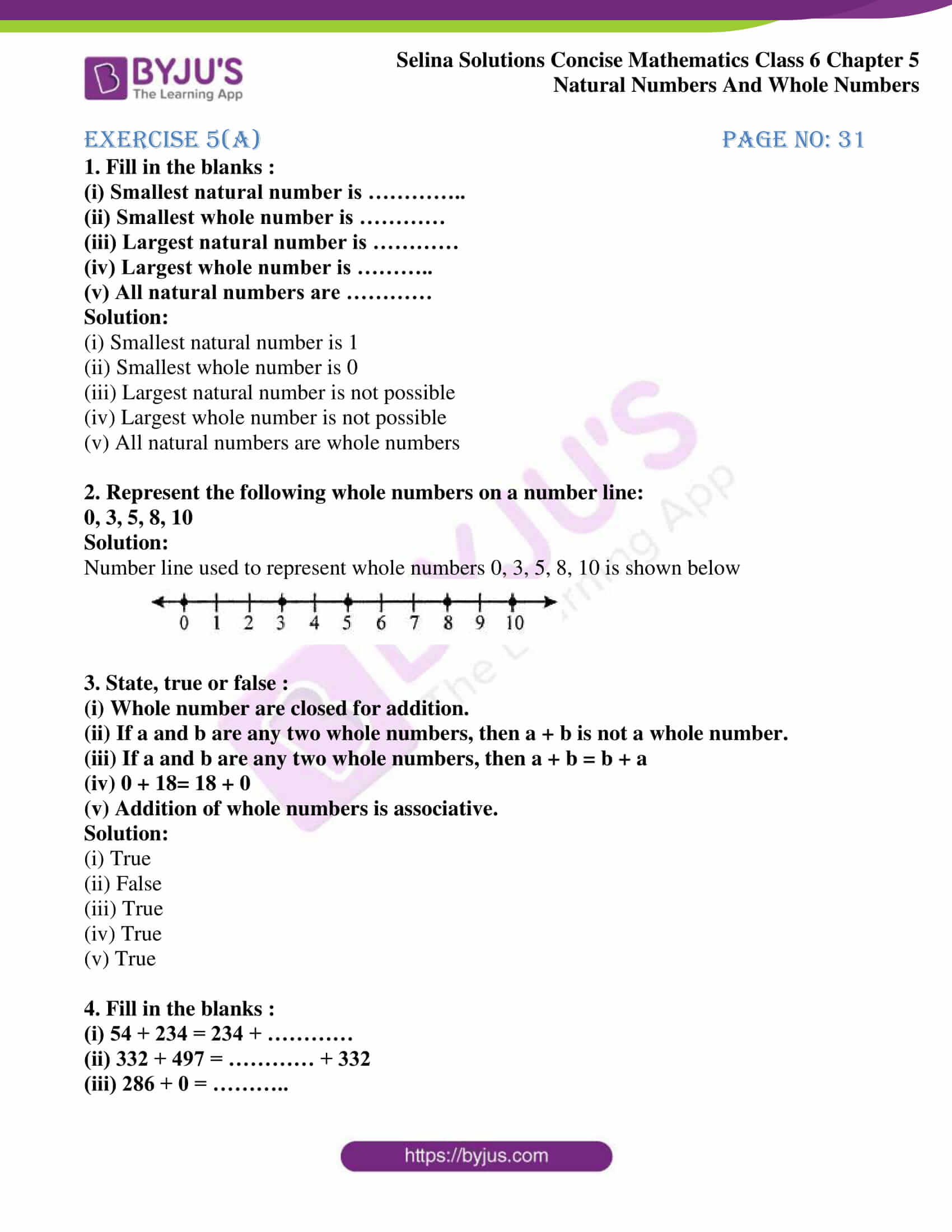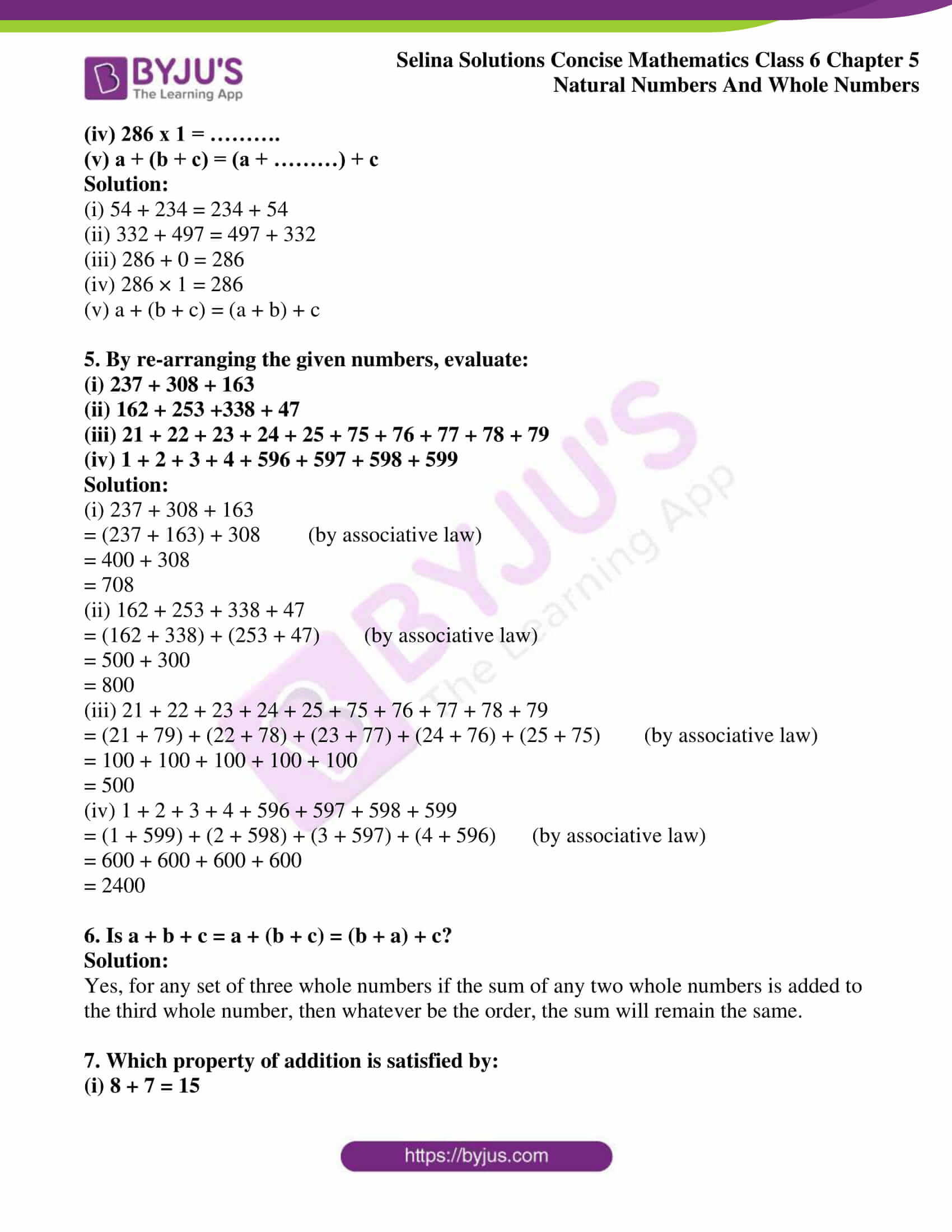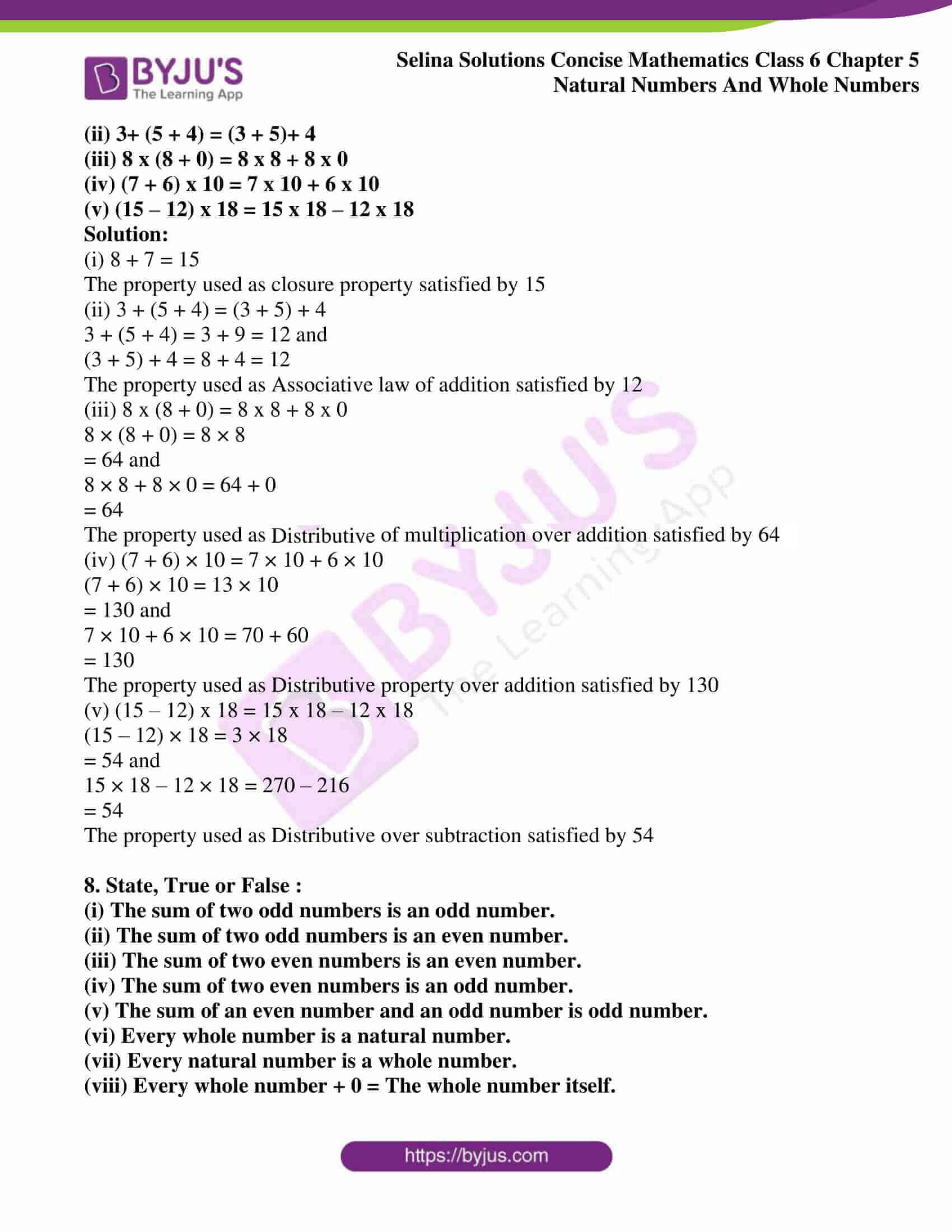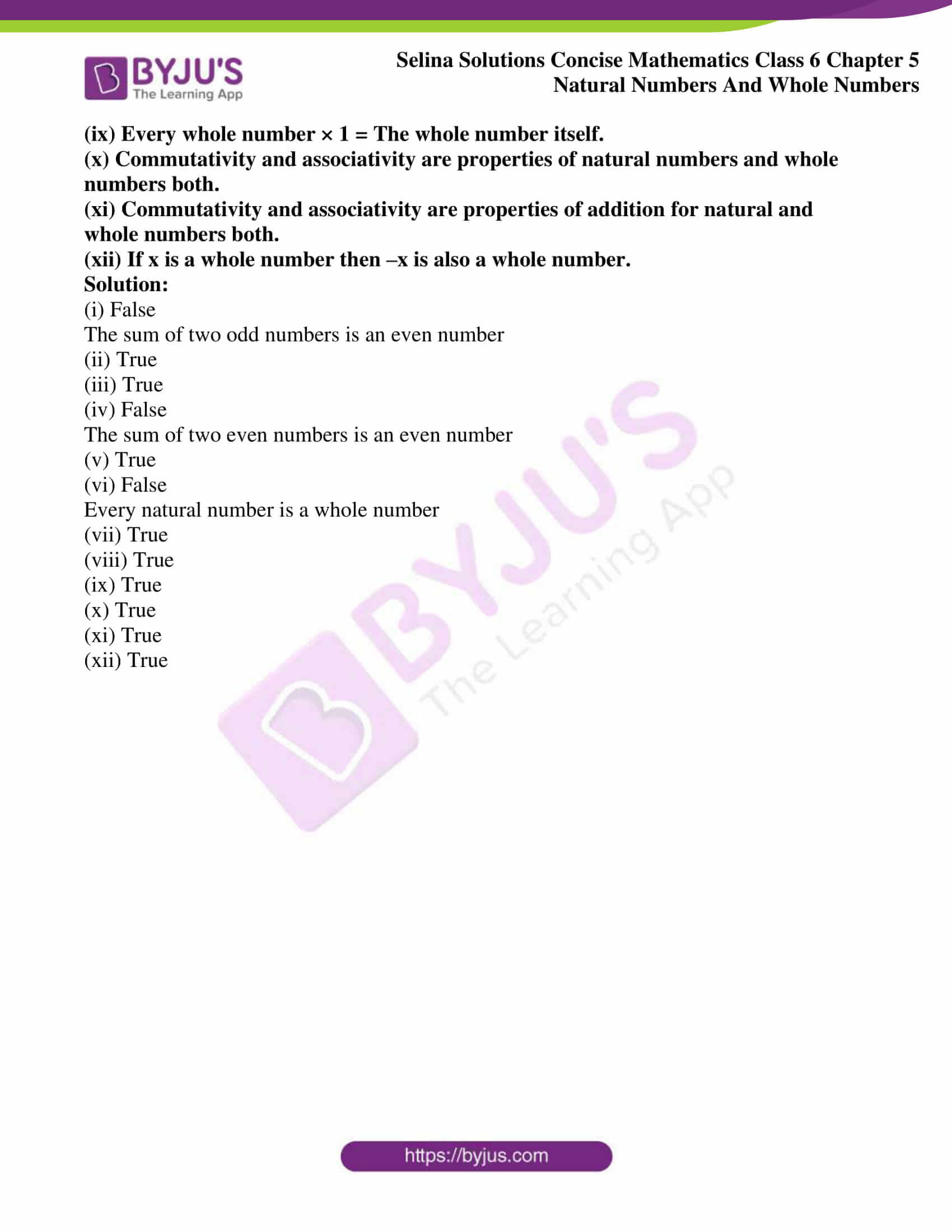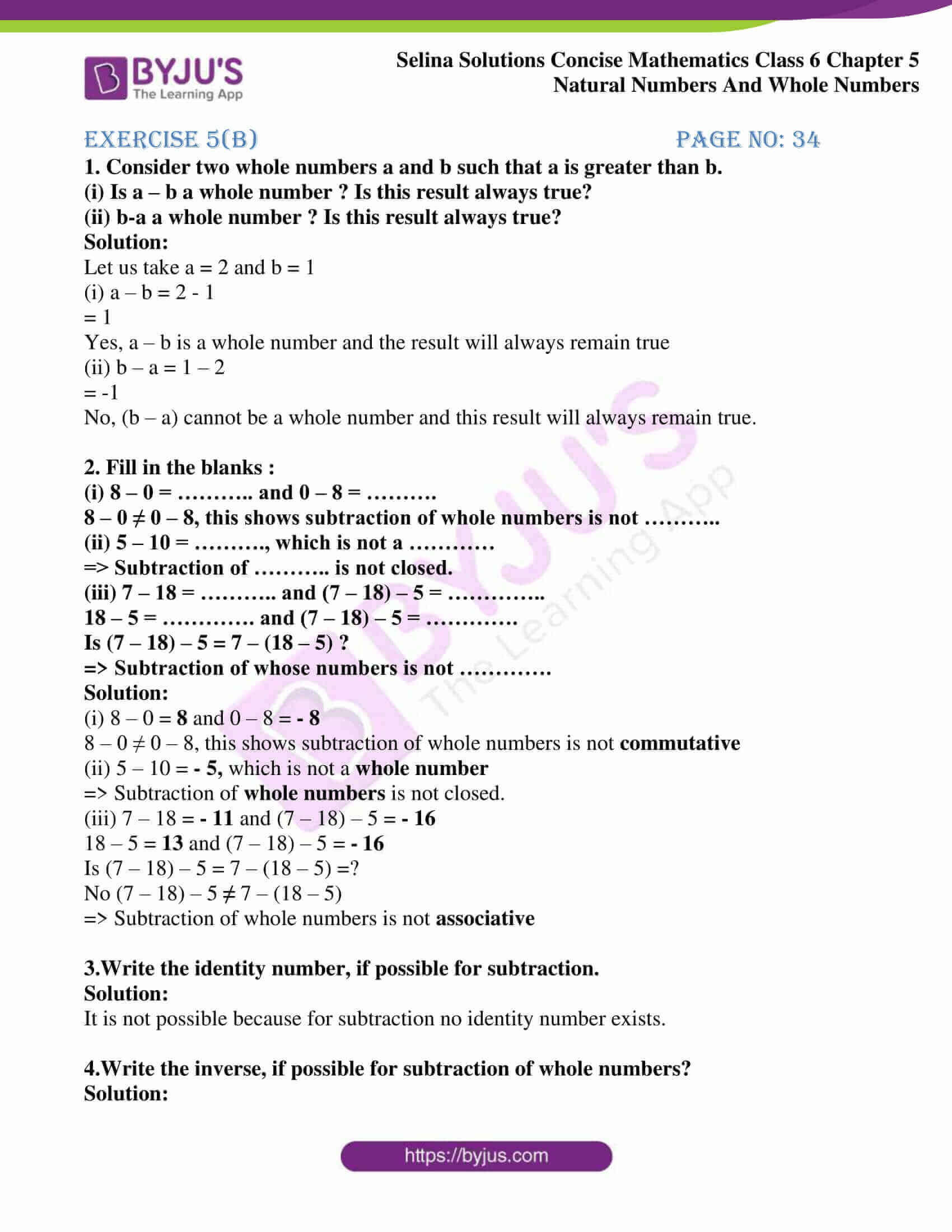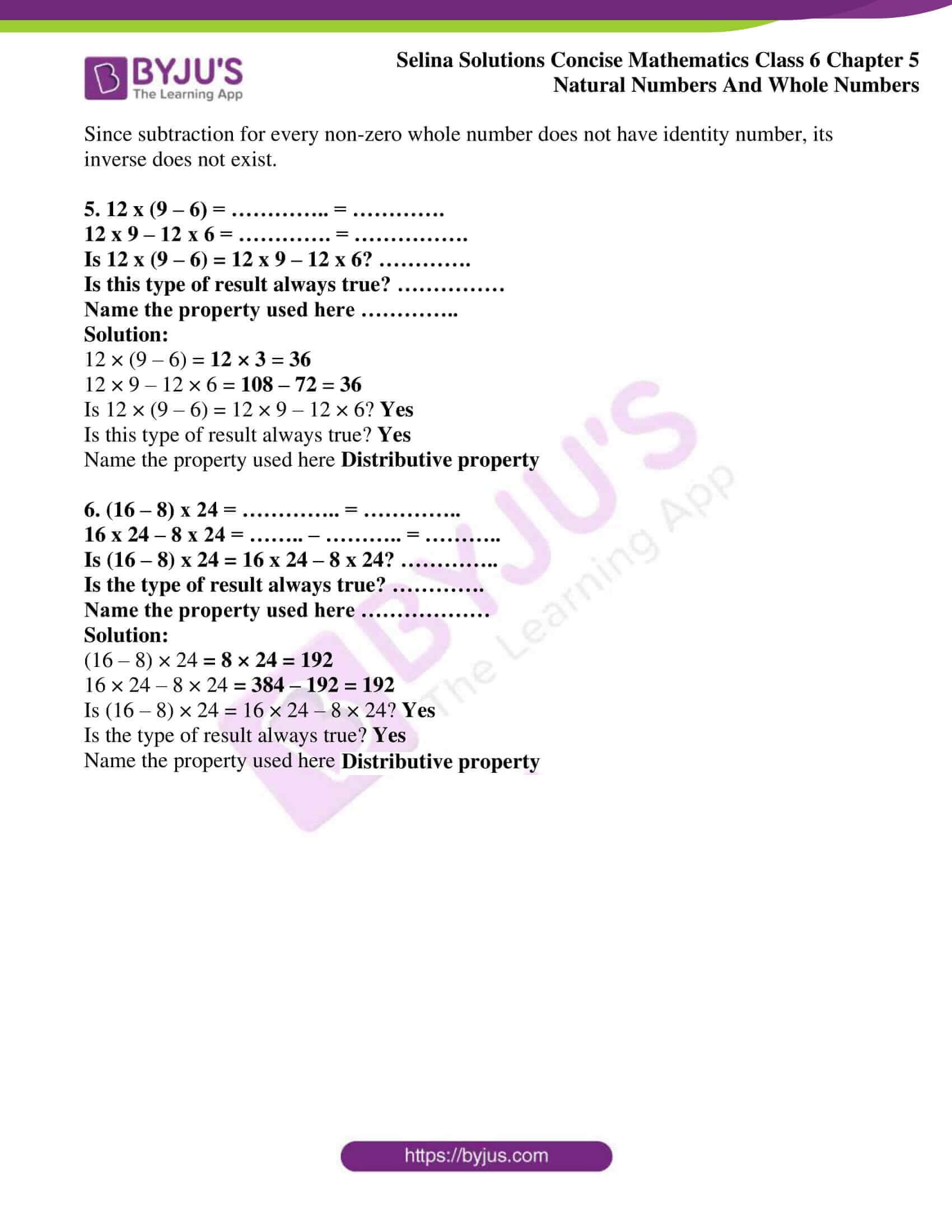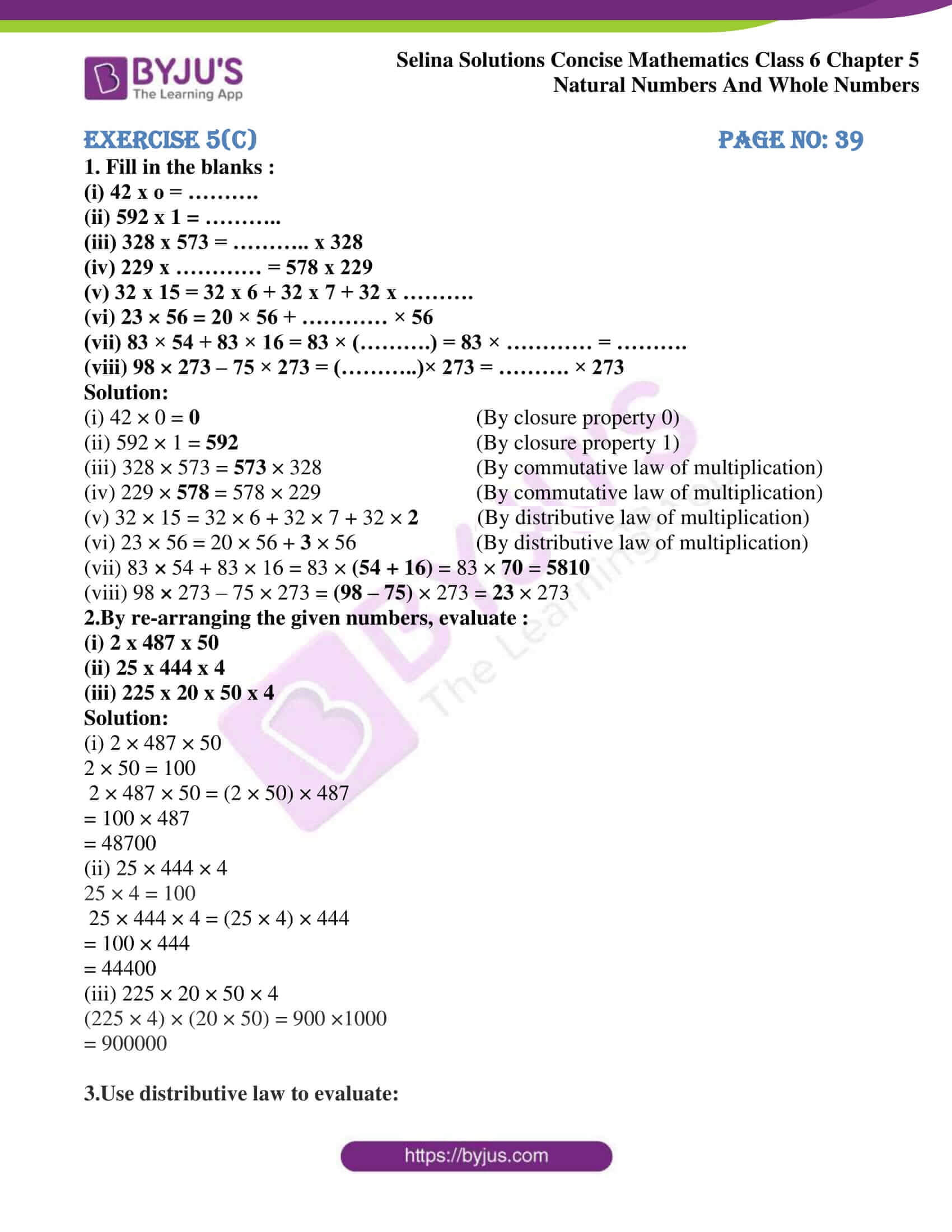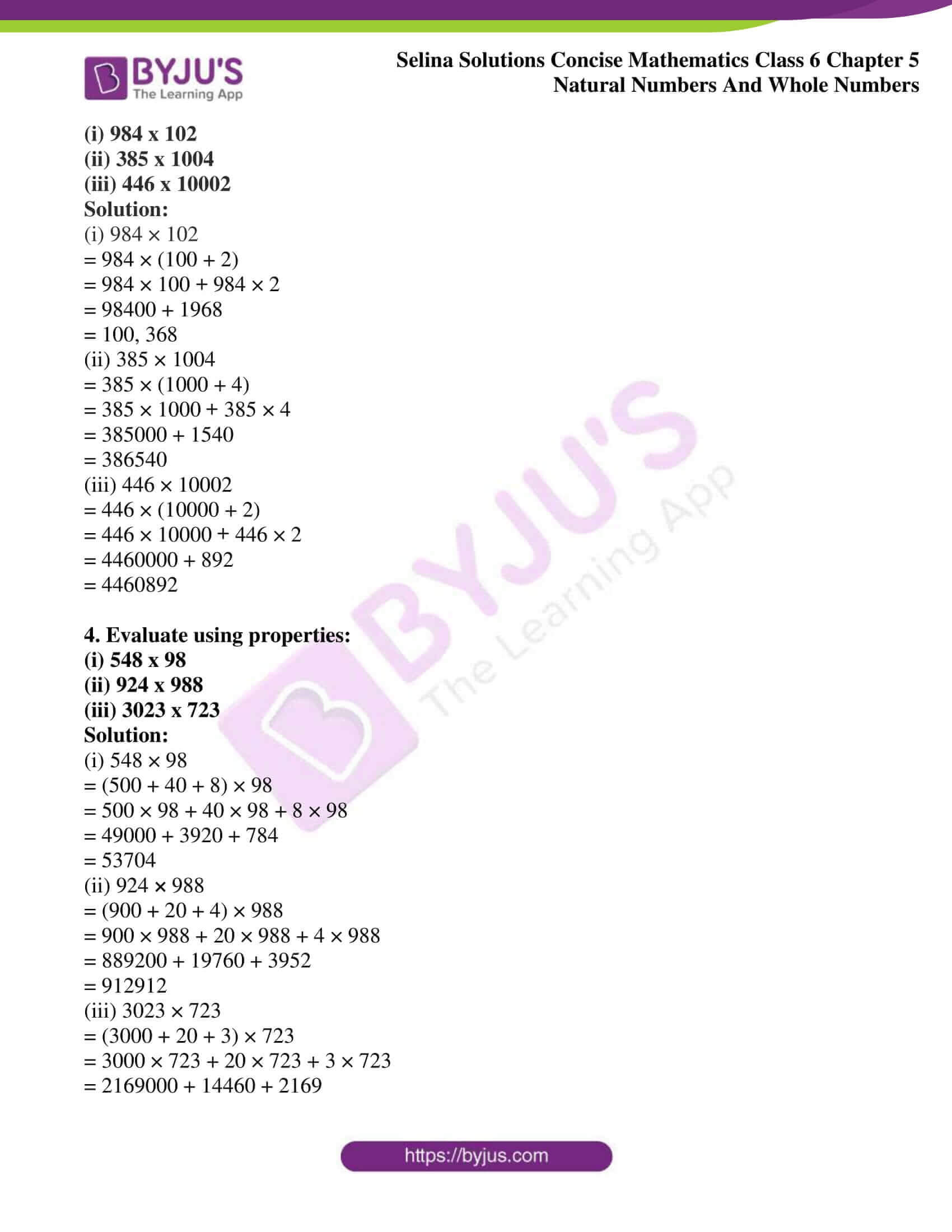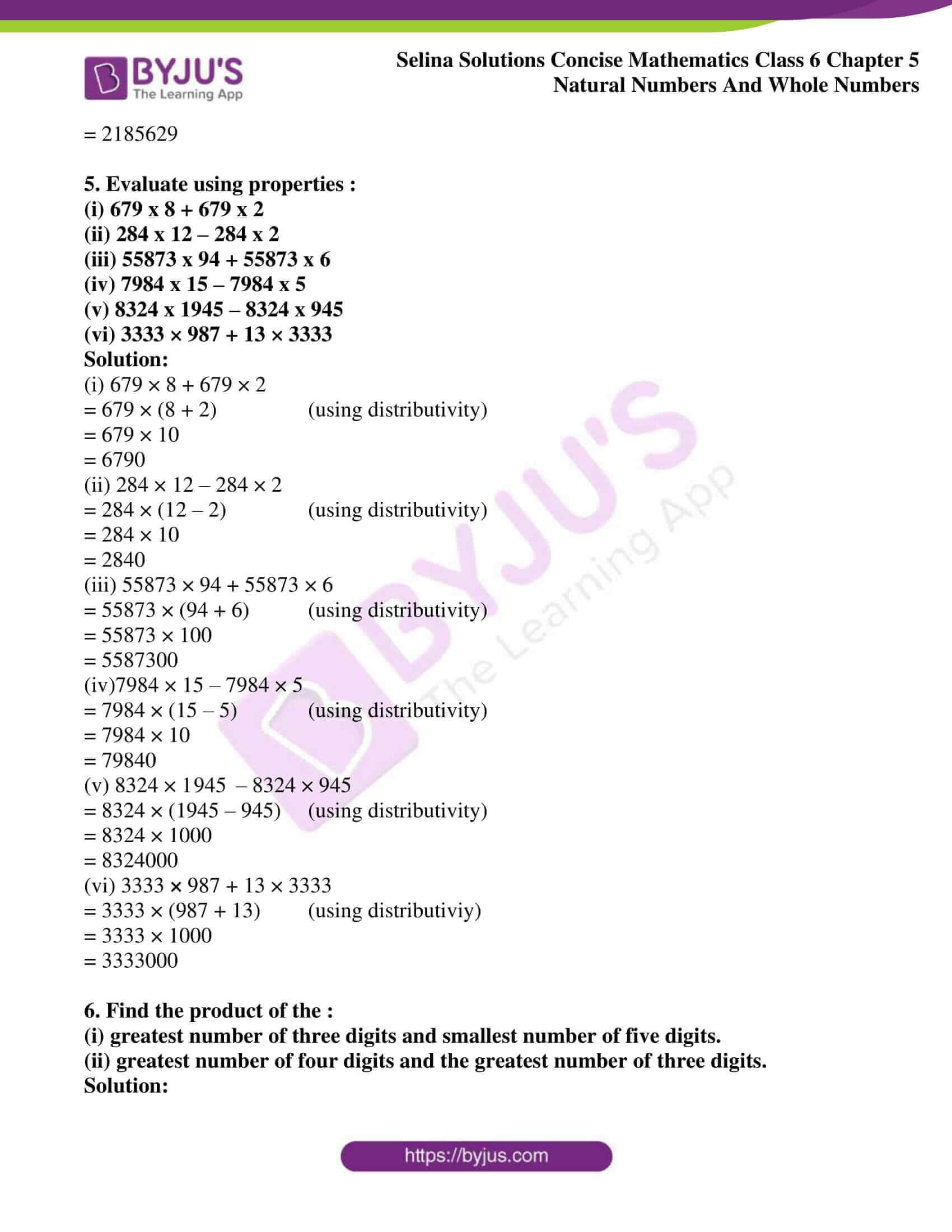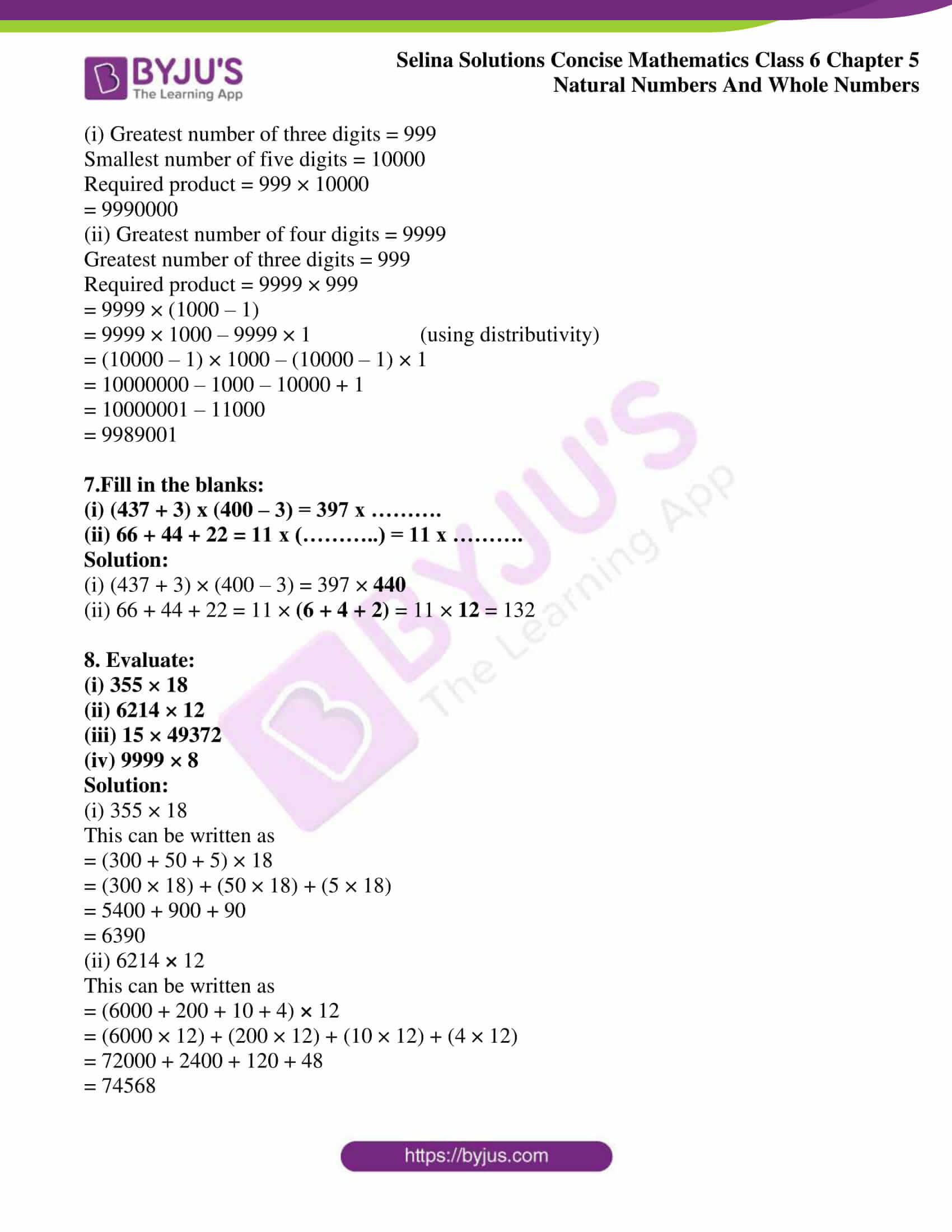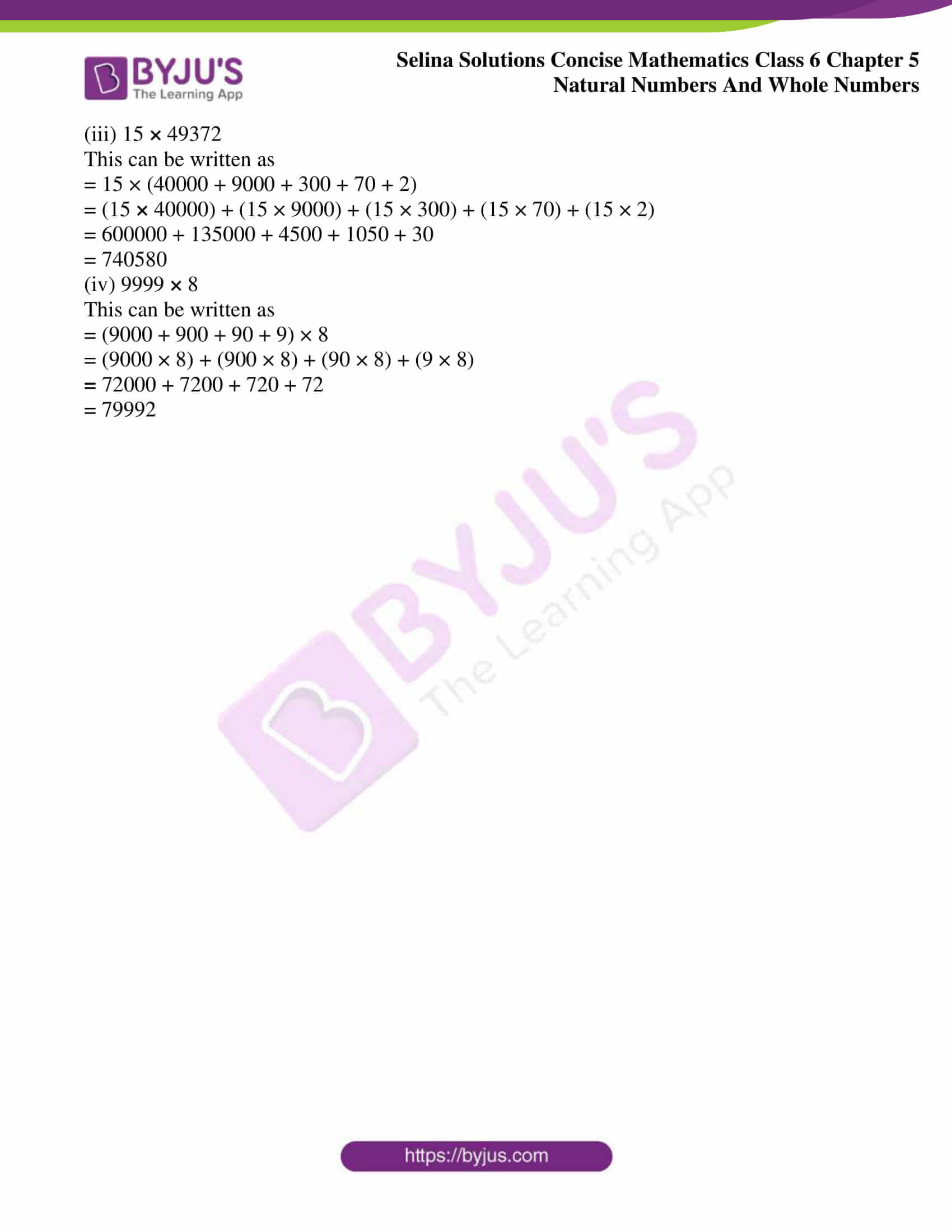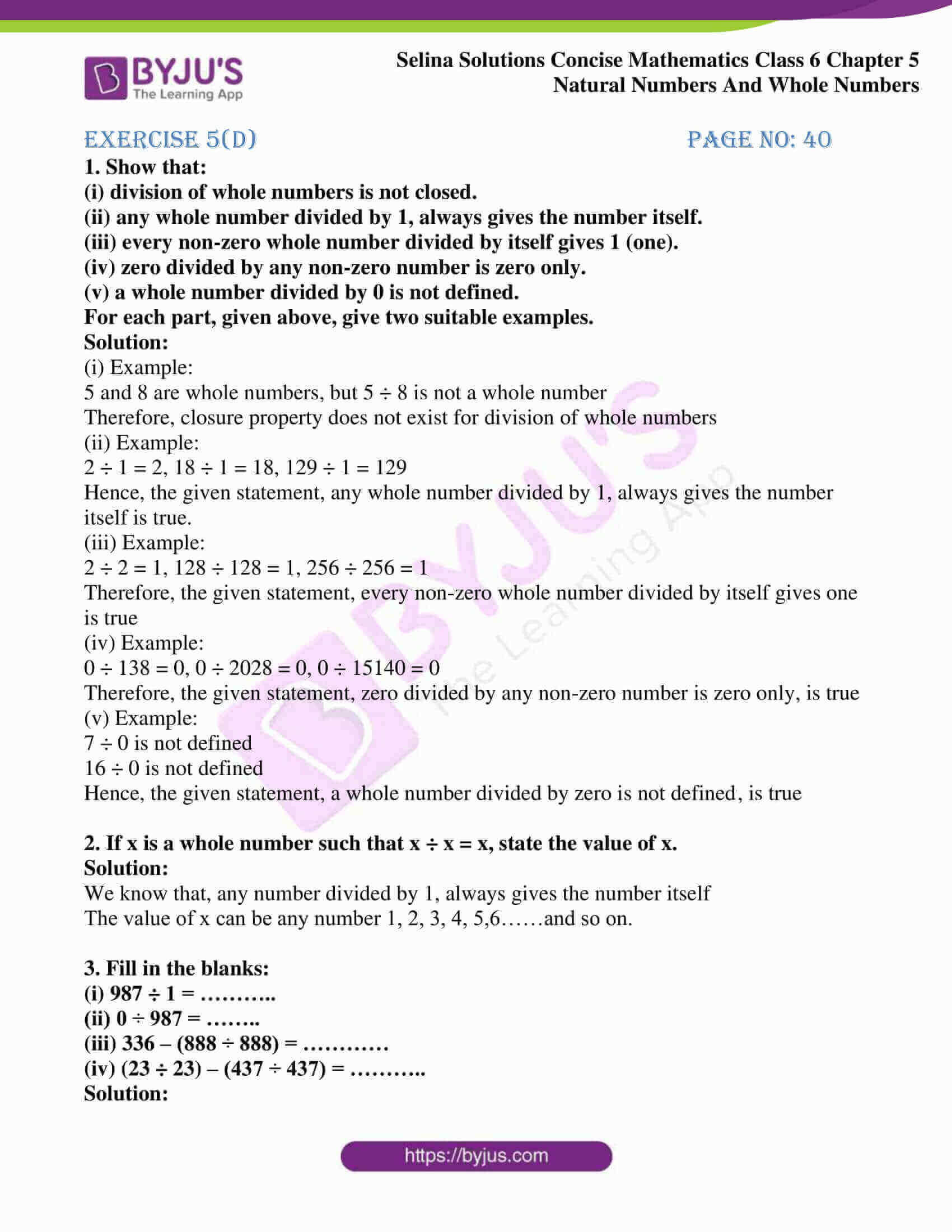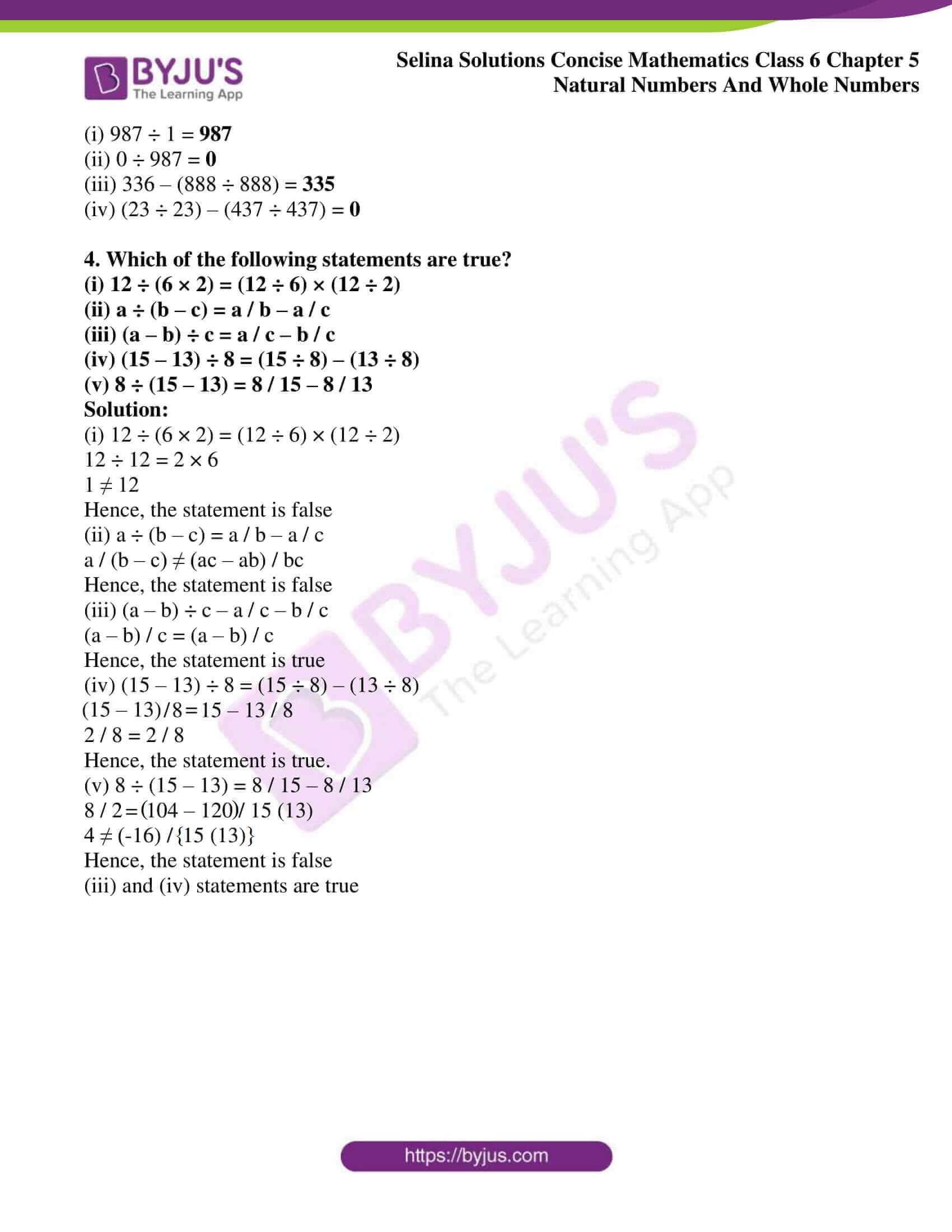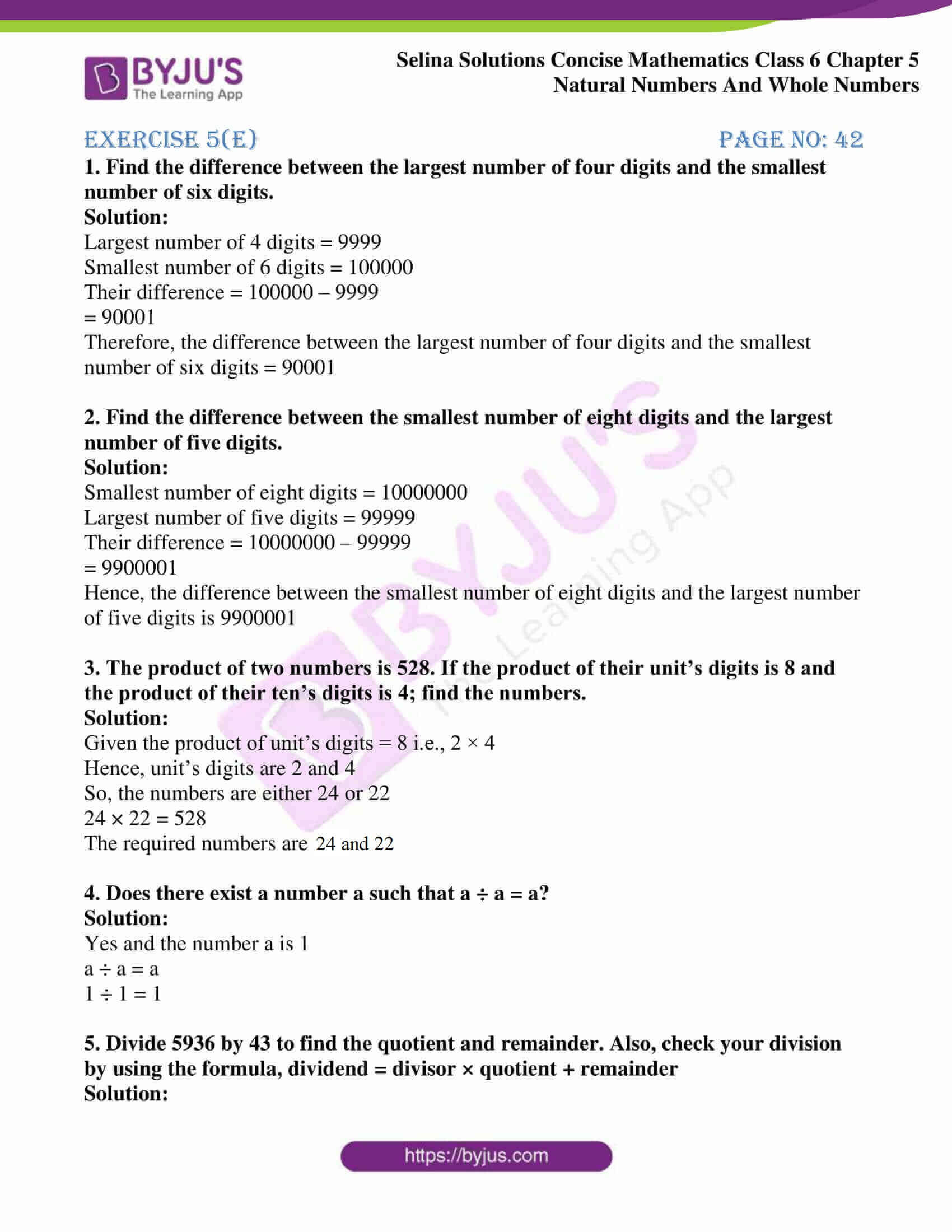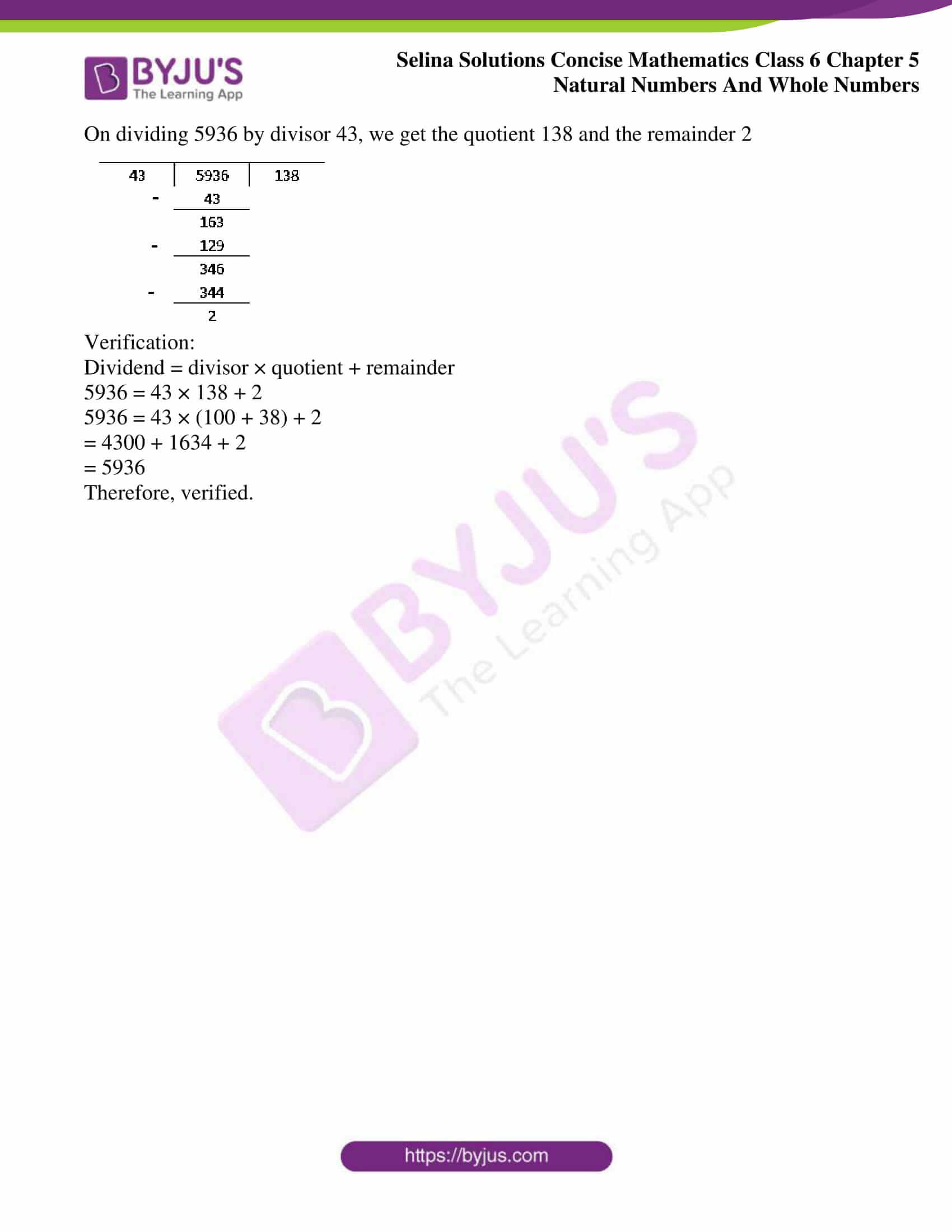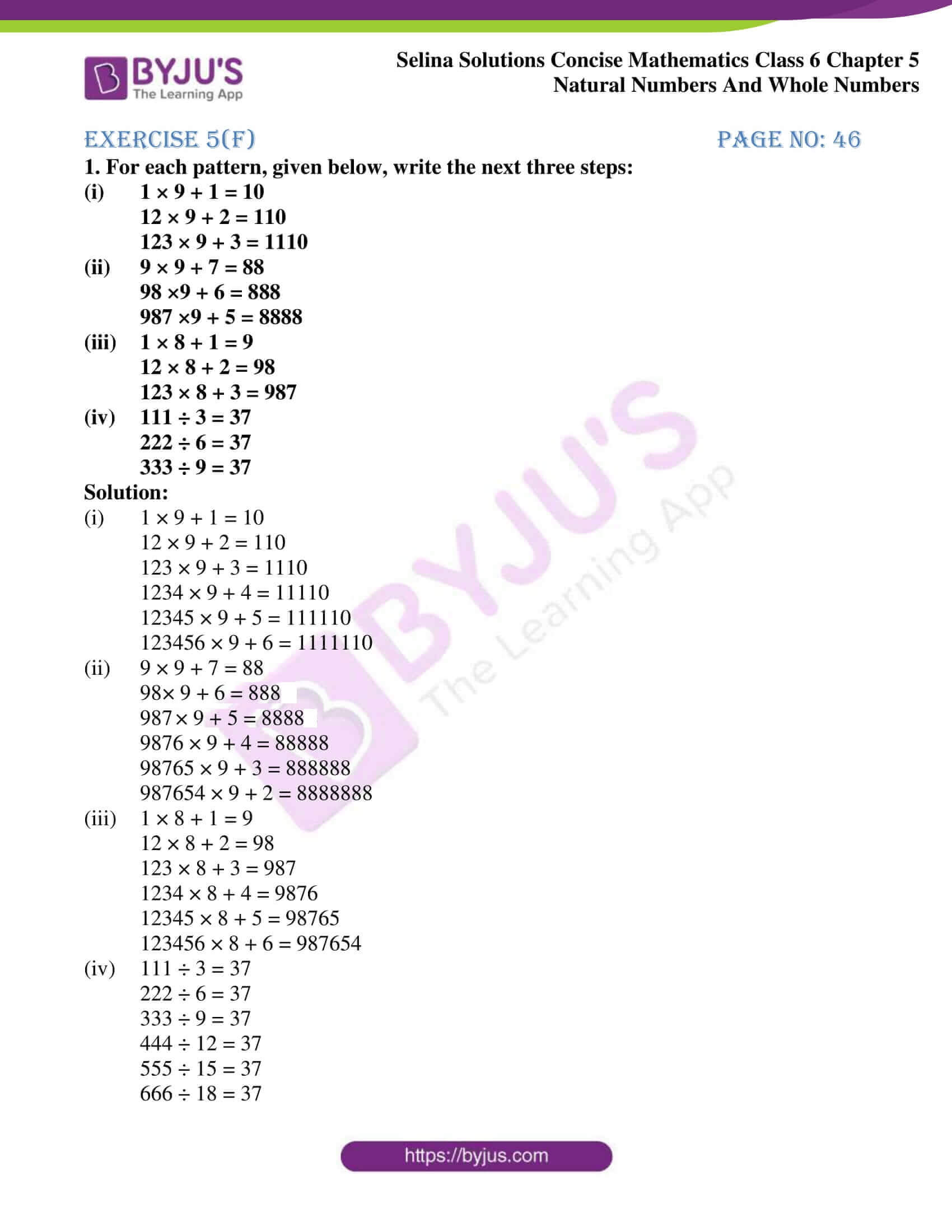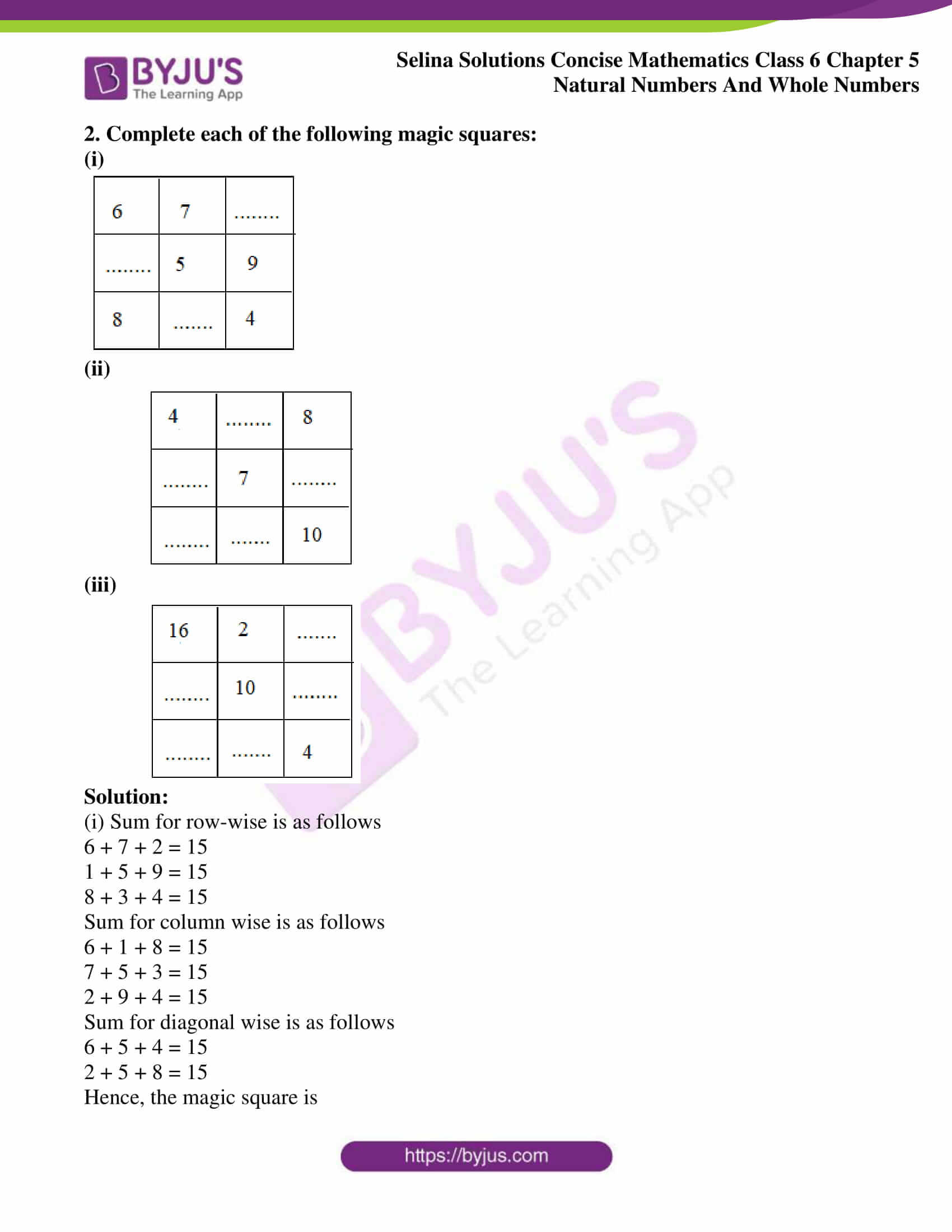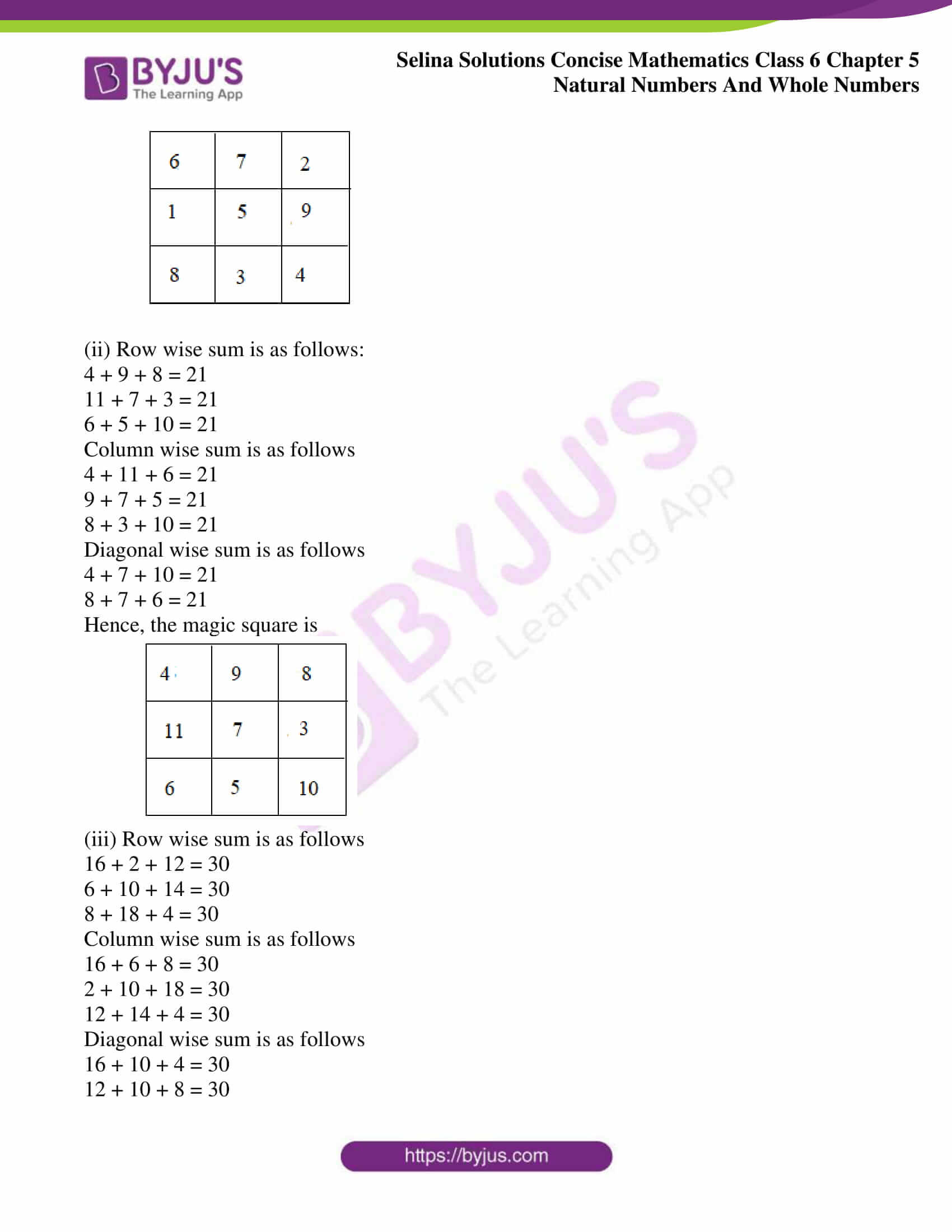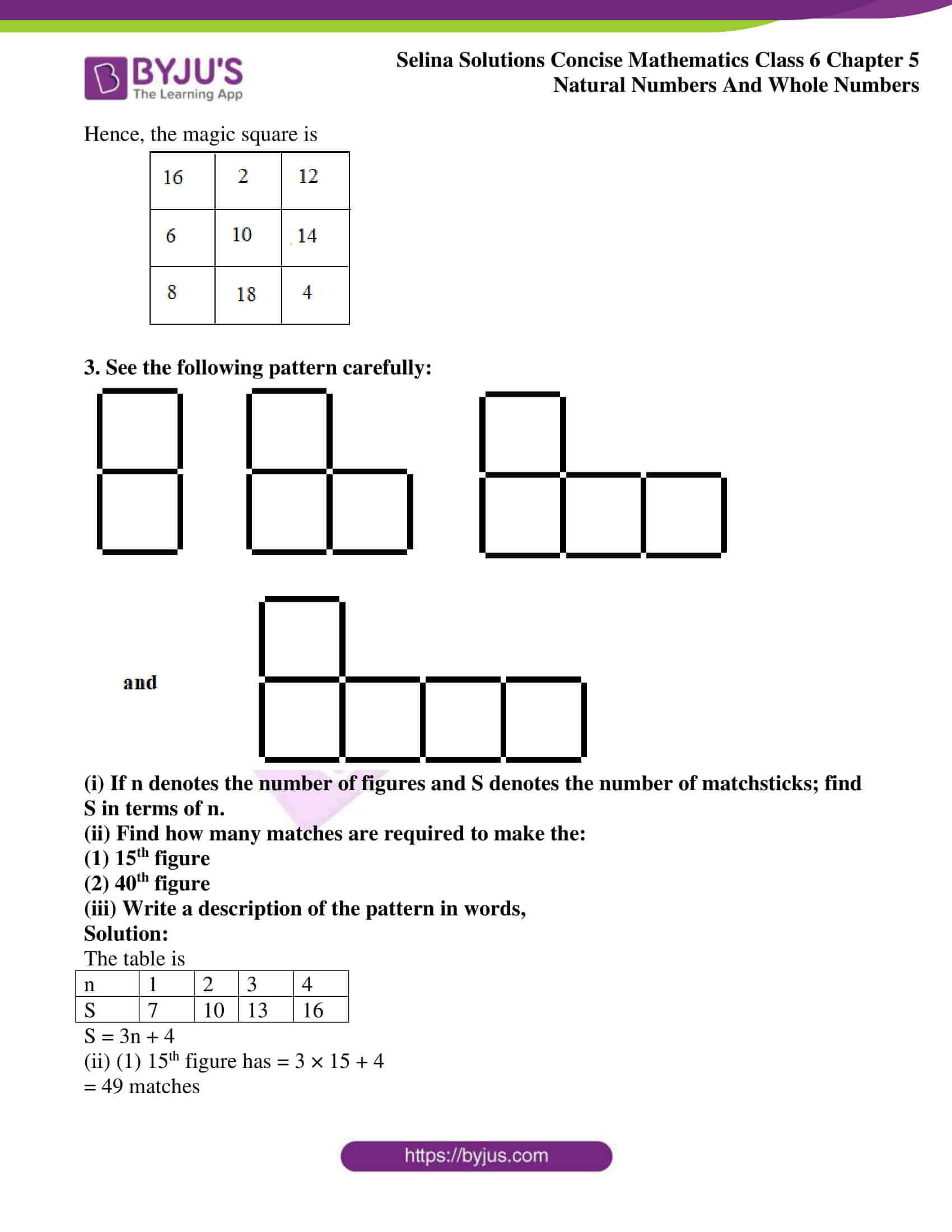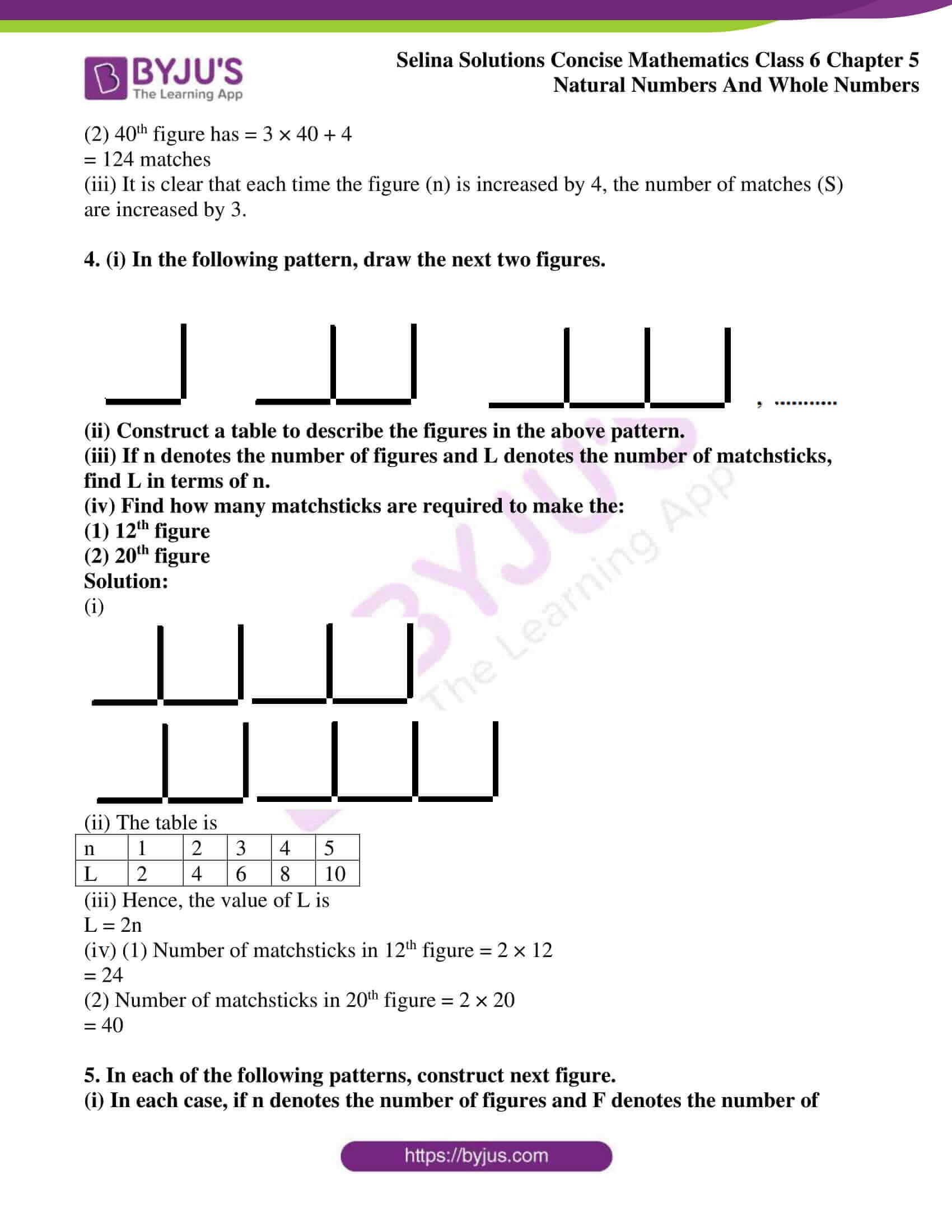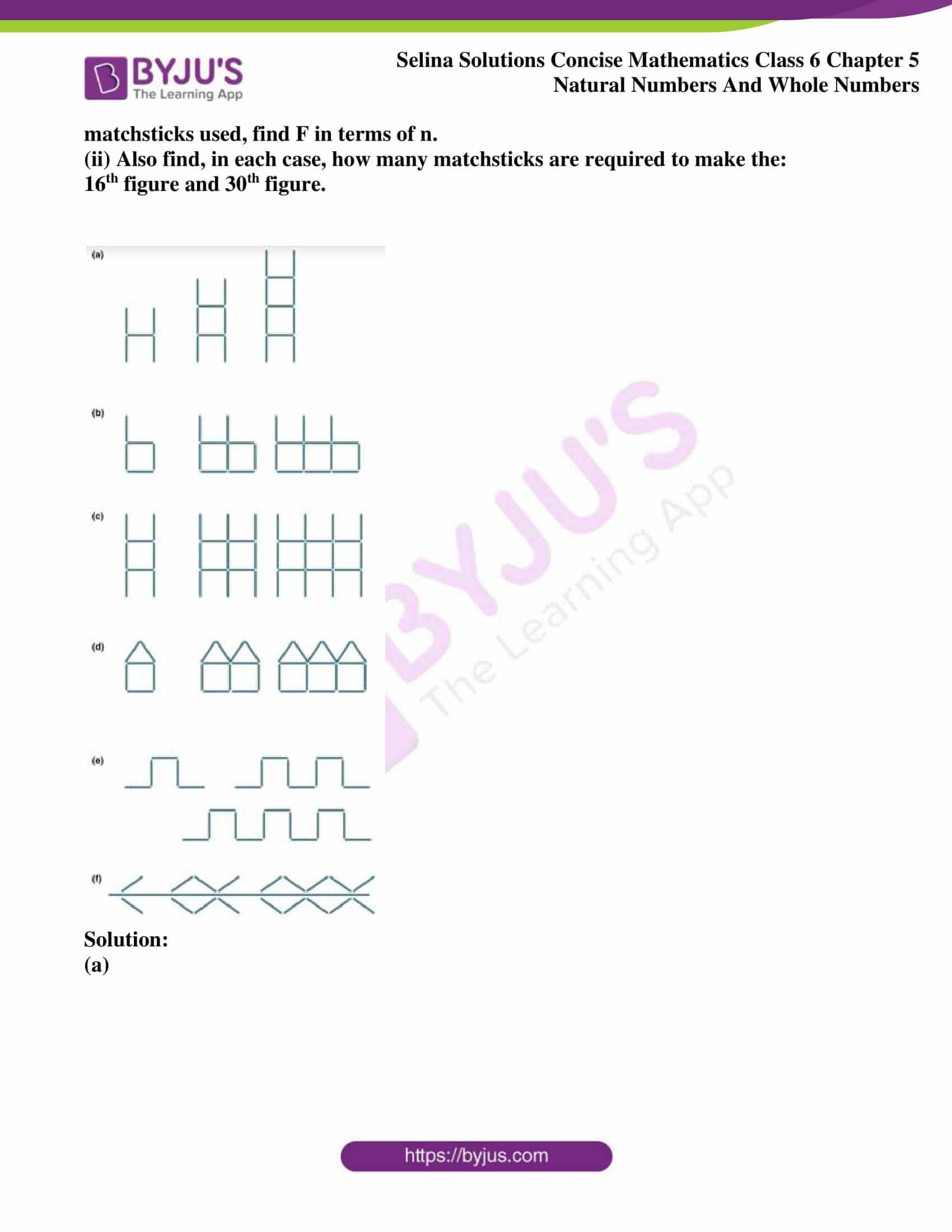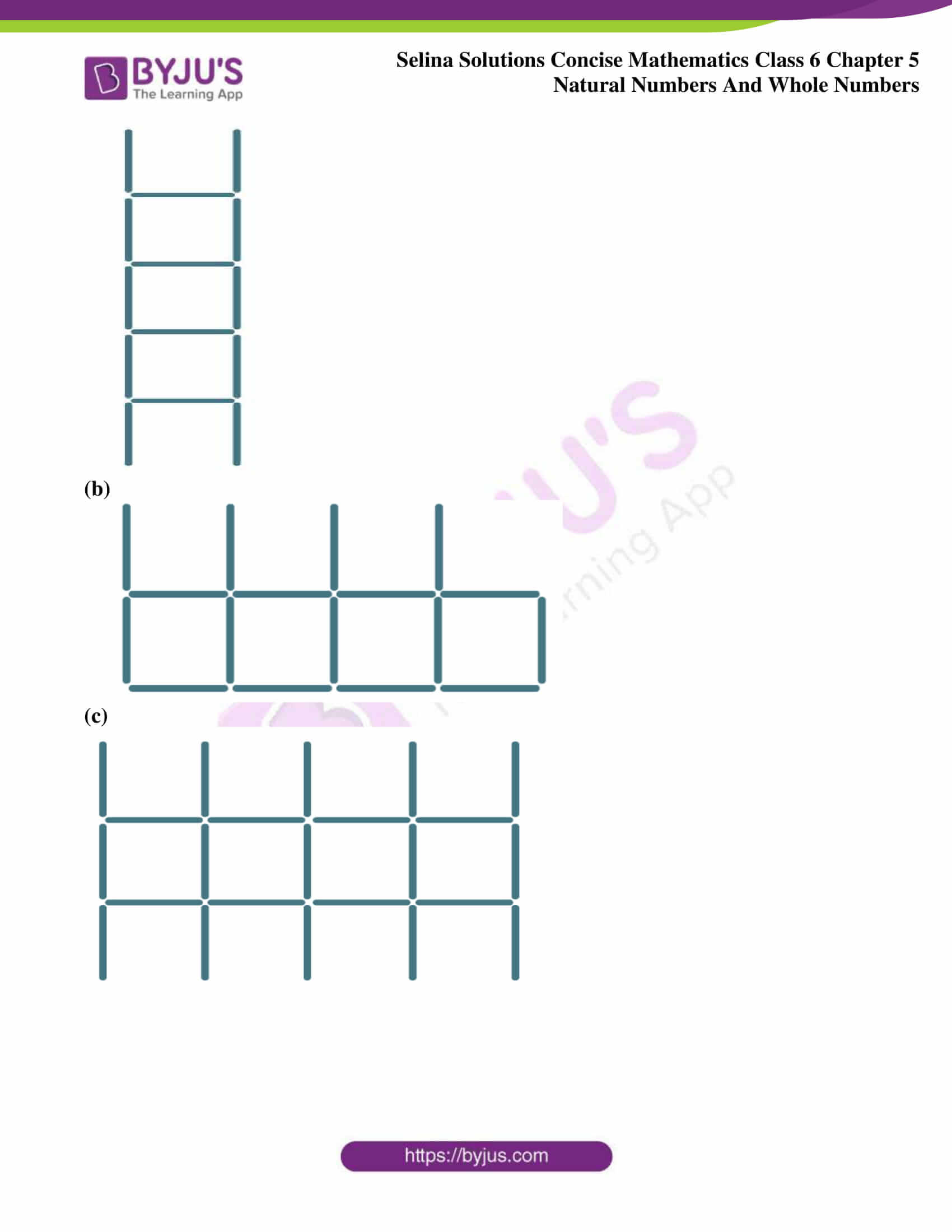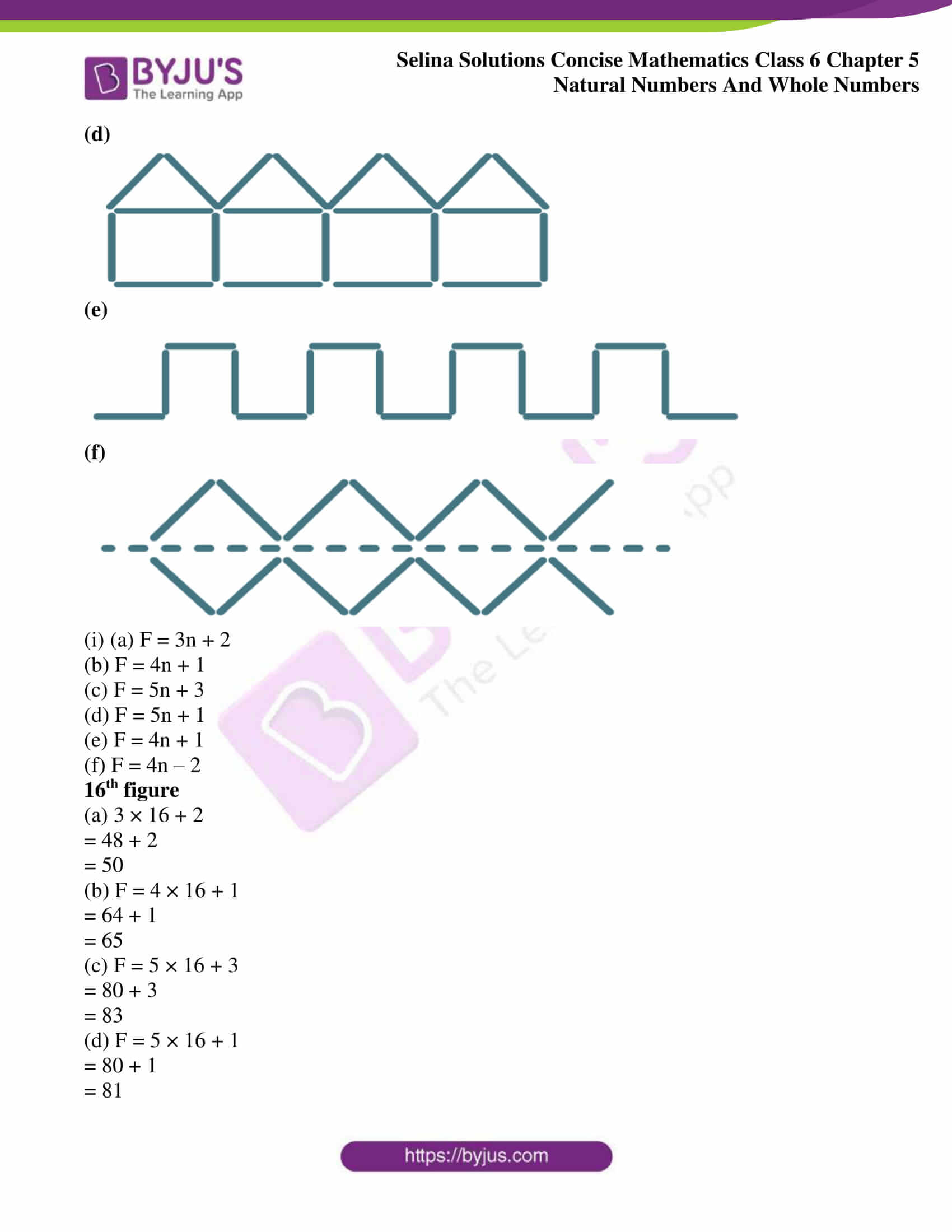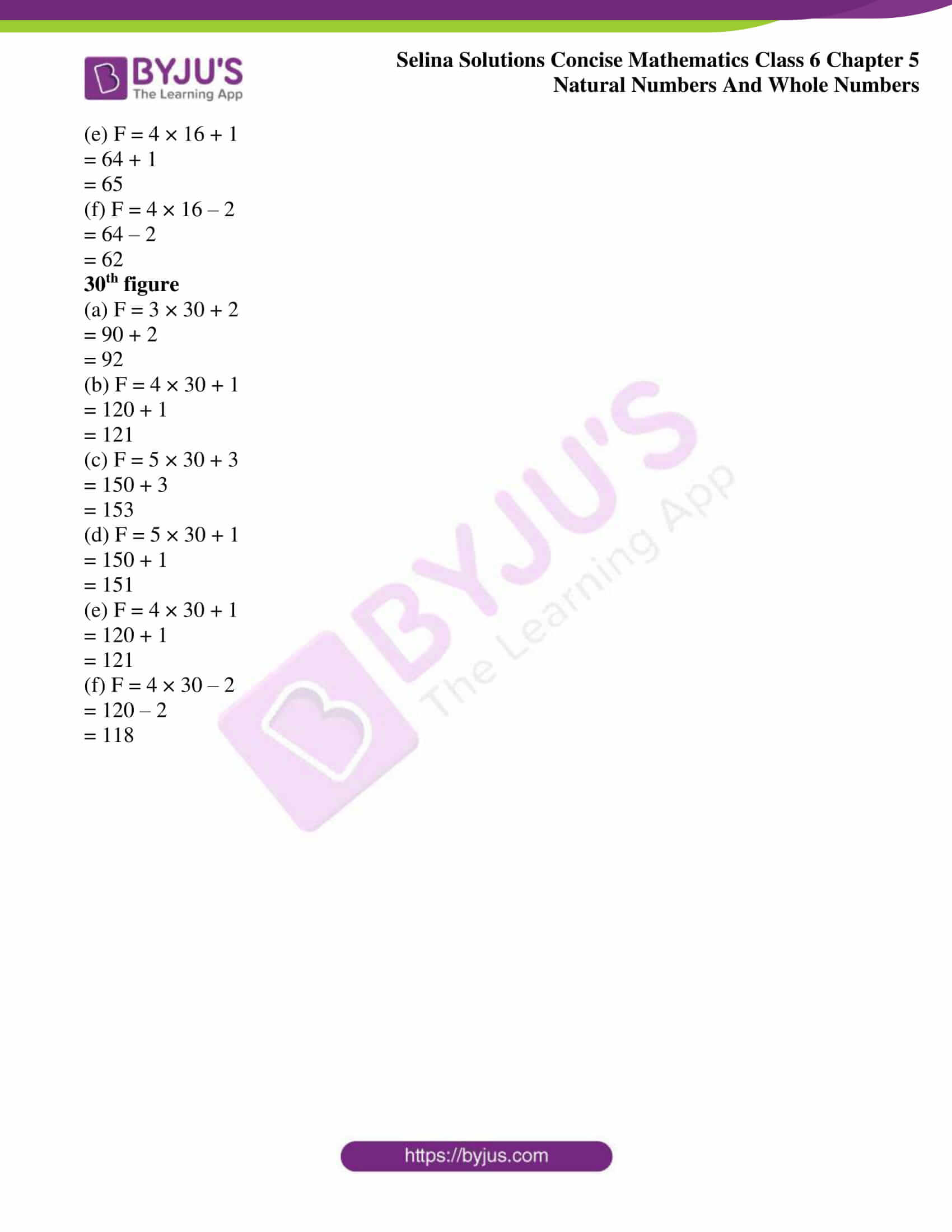## Exercises of Selina Solutions Concise Mathematics Class 6 Chapter 5 Natural Numbers And Whole Numbers

Exercise 5(A) Solutions

Exercise 5(B) Solutions

Exercise 5(C) Solutions

Exercise 5(D) Solutions

Exercise 5(E) Solutions

Exercise 5(F) Solutions

### Access Selina Solutions Concise Mathematics Class 6 Chapter 5 Natural Numbers And Whole Numbers

#### Exercise 5(A) page no: 31

1. Fill in the blanks :
(i) Smallest natural number is …………..
(ii) Smallest whole number is …………
(iii) Largest natural number is …………
(iv) Largest whole number is ………..
(v) All natural numbers are …………

Solution:

(i) Smallest natural number is 1

(ii) Smallest whole number is 0

(iii) Largest natural number is not possible

(iv) Largest whole number is not possible

(v) All natural numbers are whole numbers

2. Represent the following whole numbers on a number line:
0, 3, 5, 8, 10

Solution:

Number line used to represent whole numbers 0, 3, 5, 8, 10 is shown below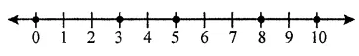3. State, true or false :
(i) Whole number are closed for addition.
(ii) If a and b are any two whole numbers, then a + b is not a whole number.
(iii) If a and b are any two whole numbers, then a + b = b + a
(iv) 0 + 18= 18 + 0
(v) Addition of whole numbers is associative.
Solution:

(i) True

(ii) False

(iii) True

(iv) True

(v) True

4. Fill in the blanks :
(i) 54 + 234 = 234 + …………
(ii) 332 + 497 = ………… + 332
(iii) 286 + 0 = ………..
(iv) 286 x 1 = ……….
(v) a + (b + c) = (a + ………) + c

Solution:

(i) 54 + 234 = 234 + 54

(ii) 332 + 497 = 497 + 332

(iii) 286 + 0 = 286

(iv) 286 × 1 = 286

(v) a + (b + c) = (a + b) + c

5. By re-arranging the given numbers, evaluate:
(i) 237 + 308 + 163
(ii) 162 + 253 +338 + 47
(iii) 21 + 22 + 23 + 24 + 25 + 75 + 76 + 77 + 78 + 79
(iv) 1 + 2 + 3 + 4 + 596 + 597 + 598 + 599

Solution:

(i) 237 + 308 + 163

= (237 + 163) + 308 (by associative law)

= 400 + 308

= 708

(ii) 162 + 253 + 338 + 47

= (162 + 338) + (253 + 47) (by associative law)

= 500 + 300

= 800

(iii) 21 + 22 + 23 + 24 + 25 + 75 + 76 + 77 + 78 + 79

= (21 + 79) + (22 + 78) + (23 + 77) + (24 + 76) + (25 + 75) (by associative law)

= 100 + 100 + 100 + 100 + 100

= 500

(iv) 1 + 2 + 3 + 4 + 596 + 597 + 598 + 599

= (1 + 599) + (2 + 598) + (3 + 597) + (4 + 596) (by associative law)

= 600 + 600 + 600 + 600

= 2400

6. Is a + b + c = a + (b + c) = (b + a) + c?

Solution:

Yes, for any set of three whole numbers if the sum of any two whole numbers is added to the third whole number, then whatever be the order, the sum will remain the same.

7. Which property of addition is satisfied by:
(i) 8 + 7 = 15
(ii) 3+ (5 + 4) = (3 + 5)+ 4
(iii) 8 x (8 + 0) = 8 x 8 + 8 x 0
(iv) (7 + 6) x 10 = 7 x 10 + 6 x 10
(v) (15 – 12) x 18 = 15 x 18 – 12 x 18

Solution:

(i) 8 + 7 = 15

The property used as closure property satisfied by 15

(ii) 3 + (5 + 4) = (3 + 5) + 4

3 + (5 + 4) = 3 + 9 = 12 and

(3 + 5) + 4 = 8 + 4 = 12

The property used as Associative law of addition satisfied by 12

(iii) 8 x (8 + 0) = 8 x 8 + 8 x 0

8 × (8 + 0) = 8 × 8

= 64 and

8 × 8 + 8 × 0 = 64 + 0

= 64

The property used as Distributive of multiplication over addition satisfied by 64

(iv) (7 + 6) × 10 = 7 × 10 + 6 × 10

(7 + 6) × 10 = 13 × 10

= 130 and

7 × 10 + 6 × 10 = 70 + 60

= 130

The property used as Distributive property over addition satisfied by 130

(v) (15 – 12) x 18 = 15 x 18 – 12 x 18

(15 – 12) × 18 = 3 × 18

= 54 and

15 × 18 – 12 × 18 = 270 – 216

= 54

The property used as Distributive over subtraction satisfied by 54

8. State, True or False :
(i) The sum of two odd numbers is an odd number.
(ii) The sum of two odd numbers is an even number.
(iii) The sum of two even numbers is an even number.
(iv) The sum of two even numbers is an odd number.
(v) The sum of an even number and an odd number is odd number.

(vi) Every whole number is a natural number.

(vii) Every natural number is a whole number.

(viii) Every whole number + 0 = The whole number itself.

(ix) Every whole number × 1 = The whole number itself.

(x) Commutativity and associativity are properties of natural numbers and whole numbers both.

(xi) Commutativity and associativity are properties of addition for natural and whole numbers both.

(xii) If x is a whole number then –x is also a whole number.

Solution:

(i) False

The sum of two odd numbers is an even number

(ii) True

(iii) True

(iv) False

The sum of two even numbers is an even number

(v) True

(vi) False

Every natural number is a whole number

(vii) True

(viii) True

(ix) True

(x) True

(xi) True

(xii) True

#### Exercise 5(b) page no: 34

1. Consider two whole numbers a and b such that a is greater than b.
(i) Is a – b a whole number ? Is this result always true?
(ii) b-a a whole number ? Is this result always true?

Solution:

Let us take a = 2 and b = 1

(i) a – b = 2 – 1

= 1

Yes, a – b is a whole number and the result will always remain true

(ii) b – a = 1 – 2

= -1

No, (b – a) cannot be a whole number and this result will always remain true.

2. Fill in the blanks :
(i) 8 – 0 = ……….. and 0 – 8 = ……….
8 – 0 ≠ 0 – 8, this shows subtraction of whole numbers is not ………..
(ii) 5 – 10 = ………., which is not a …………
=> Subtraction of ……….. is not closed.
(iii) 7 – 18 = ……….. and (7 – 18) – 5 = …………..
18 – 5 = …………. and (7 – 18) – 5 = ………….
Is (7 – 18) – 5 = 7 – (18 – 5) ?
=> Subtraction of whose numbers is not ………….

Solution:

(i) 8 – 0 = 8 and 0 – 8 = – 8

8 – 0 ≠ 0 – 8, this shows subtraction of whole numbers is not commutative

(ii) 5 – 10 = – 5, which is not a whole number

=> Subtraction of whole numbers is not closed.

(iii) 7 – 18 = – 11 and (7 – 18) – 5 = – 16

18 – 5 = 13 and (7 – 18) – 5 = – 16

Is (7 – 18) – 5 = 7 – (18 – 5) =?

No (7 – 18) – 5 7 – (18 – 5)

=> Subtraction of whole numbers is not associative

3.Write the identity number, if possible for subtraction.

Solution:

It is not possible because for subtraction no identity number exists.

4.Write the inverse, if possible for subtraction of whole numbers?

Solution:

Since subtraction for every non-zero whole number does not have identity number, its inverse does not exist.

5. 12 x (9 – 6) = ………….. = ………….
12 x 9 – 12 x 6 = …………. = …………….
Is 12 x (9 – 6) = 12 x 9 – 12 x 6? ………….
Is this type of result always true? ……………
Name the property used here …………..

Solution:

12 × (9 – 6) = 12 × 3 = 36

12 × 9 – 12 × 6 = 108 – 72 = 36

Is 12 × (9 – 6) = 12 × 9 – 12 × 6? Yes

Is this type of result always true? Yes

Name the property used here Distributive property

6. (16 – 8) x 24 = ………….. = …………..
16 x 24 – 8 x 24 = …….. – ……….. = ………..
Is (16 – 8) x 24 = 16 x 24 – 8 x 24? …………..
Is the type of result always true? ………….
Name the property used here ………………

Solution:

(16 – 8) × 24 = 8 × 24 = 192

16 × 24 – 8 × 24 = 384 – 192 = 192

Is (16 – 8) × 24 = 16 × 24 – 8 × 24? Yes

Is the type of result always true? Yes

Name the property used here Distributive property.

#### Exercise 5(c) page no: 39

1. Fill in the blanks :
(i) 42 x o = ……….
(ii) 592 x 1 = ………..
(iii) 328 x 573 = ……….. x 328
(iv) 229 x ………… = 578 x 229
(v) 32 x 15 = 32 x 6 + 32 x 7 + 32 x ……….

(vi) 23 × 56 = 20 × 56 + ………… × 56

(vii) 83 × 54 + 83 × 16 = 83 × (……….) = 83 × ………… = ……….

(viii) 98 × 273 – 75 × 273 = (………..)× 273 = ………. × 273

Solution:

(i) 42 × 0 = 0 (By closure property 0)

(ii) 592 × 1 = 592 (By closure property 1)

(iii) 328 × 573 = 573 × 328 (By commutative law of multiplication)

(iv) 229 × 578 = 578 × 229 (By commutative law of multiplication)

(v) 32 × 15 = 32 × 6 + 32 × 7 + 32 × 2 (By distributive law of multiplication)

(vi) 23 × 56 = 20 × 56 + 3 × 56 (By distributive law of multiplication)

(vii) 83 × 54 + 83 × 16 = 83 × (54 + 16) = 83 × 70 = 5810

(viii) 98 × 273 – 75 × 273 = (98 – 75) × 273 = 23 × 273

2.By re-arranging the given numbers, evaluate :
(i) 2 x 487 x 50
(ii) 25 x 444 x 4
(iii) 225 x 20 x 50 x 4

Solution:

(i) 2 × 487 × 50

2 × 50 = 100

2 × 487 × 50 = (2 × 50) × 487

= 100 × 487

= 48700

(ii) 25 × 444 × 4

25 × 4 = 100

25 × 444 × 4 = (25 × 4) × 444

= 100 × 444

= 44400

(iii) 225 × 20 × 50 × 4

(225 × 4) × (20 × 50) = 900 ×1000

= 900000

3.Use distributive law to evaluate:
(i) 984 x 102
(ii) 385 x 1004
(iii) 446 x 10002

Solution:

(i) 984 × 102

= 984 × (100 + 2)

= 984 × 100 + 984 × 2

= 98400 + 1968

= 100, 368

(ii) 385 × 1004

= 385 × (1000 + 4)

= 385 × 1000 + 385 × 4

= 385000 + 1540

= 386540

(iii) 446 × 10002

= 446 × (10000 + 2)

= 446 × 10000 + 446 × 2

= 4460000 + 892

= 4460892

4. Evaluate using properties:
(i) 548 x 98
(ii) 924 x 988
(iii) 3023 x 723

Solution:

(i) 548 × 98

= (500 + 40 + 8) × 98

= 500 × 98 + 40 × 98 + 8 × 98

= 49000 + 3920 + 784

= 53704

(ii) 924 × 988

= (900 + 20 + 4) × 988

= 900 × 988 + 20 × 988 + 4 × 988

= 889200 + 19760 + 3952

= 912912

(iii) 3023 × 723

= (3000 + 20 + 3) × 723

= 3000 × 723 + 20 × 723 + 3 × 723

= 2169000 + 14460 + 2169

= 2185629

5. Evaluate using properties :
(i) 679 x 8 + 679 x 2
(ii) 284 x 12 – 284 x 2
(iii) 55873 x 94 + 55873 x 6
(iv) 7984 x 15 – 7984 x 5
(v) 8324 x 1945 – 8324 x 945

(vi) 3333 × 987 + 13 × 3333

Solution:

(i) 679 × 8 + 679 × 2

= 679 × (8 + 2) (using distributivity)

= 679 × 10

= 6790

(ii) 284 × 12 – 284 × 2

= 284 × (12 – 2) (using distributivity)

= 284 × 10

= 2840

(iii) 55873 × 94 + 55873 × 6

= 55873 × (94 + 6) (using distributivity)

= 55873 × 100

= 5587300

(iv)7984 × 15 – 7984 × 5

= 7984 × (15 – 5) (using distributivity)

= 7984 × 10

= 79840

(v) 8324 × 1.945 – 8324 × 945

= 8324 × (1945 – 945) (using distributivity)

= 8324 × 1000

= 8324000

(vi) 3333 × 987 + 13 × 3333

= 3333 × (987 + 13) (using distributiviy)

= 3333 × 1000

= 3333000

6. Find the product of the :
(i) greatest number of three digits and smallest number of five digits.
(ii) greatest number of four digits and the greatest number of three digits.

Solution:

(i) Greatest number of three digits = 999

Smallest number of five digits = 10000

Required product = 999 × 10000

= 9990000

(ii) Greatest number of four digits = 9999

Greatest number of three digits = 999

Required product = 9999 × 999

= 9999 × (1000 – 1)

= 9999 × 1000 – 9999 × 1 (using distributivity)

= (10000 – 1) × 1000 – (10000 – 1) × 1

= 10000000 – 1000 – 10000 + 1

= 10000001 – 11000

= 9989001

7.Fill in the blanks:
(i) (437 + 3) x (400 – 3) = 397 x ……….
(ii) 66 + 44 + 22 = 11 x (………..) = 11 x ……….

Solution:

(i) (437 + 3) × (400 – 3) = 397 × 440

(ii) 66 + 44 + 22 = 11 × (6 + 4 + 2) = 11 × 12 = 132

8. Evaluate:

(i) 355 × 18

(ii) 6214 × 12

(iii) 15 × 49372

(iv) 9999 × 8

Solution:

(i) 355 × 18

This can be written as

= (300 + 50 + 5) × 18

= (300 × 18) + (50 × 18) + (5 × 18)

= 5400 + 900 + 90

= 6390

(ii) 6214 × 12

This can be written as

= (6000 + 200 + 10 + 4) × 12

= (6000 × 12) + (200 × 12) + (10 × 12) + (4 × 12)

= 72000 + 2400 + 120 + 48

= 74568

(iii) 15 × 49372

This can be written as

= 15 × (40000 + 9000 + 300 + 70 + 2)

= (15 × 40000) + (15 × 9000) + (15 × 300) + (15 × 70) + (15 × 2)

= 600000 + 135000 + 4500 + 1050 + 30

= 740580

(iv) 9999 × 8

This can be written as

= (9000 + 900 + 90 + 9) × 8

= (9000 × 8) + (900 × 8) + (90 × 8) + (9 × 8)

= 72000 + 7200 + 720 + 72

= 79992

#### Exercise 5(D) page no: 40

1. Show that:

(i) division of whole numbers is not closed.

(ii) any whole number divided by 1, always gives the number itself.

(iii) every non-zero whole number divided by itself gives 1 (one).

(iv) zero divided by any non-zero number is zero only.

(v) a whole number divided by 0 is not defined.

For each part, given above, give two suitable examples.

Solution:

(i) Example:

5 and 8 are whole numbers, but 5 ÷ 8 is not a whole number

Therefore, closure property does not exist for division of whole numbers

(ii) Example:

2 ÷ 1 = 2, 18 ÷ 1 = 18, 129 ÷ 1 = 129

Hence, the given statement, any whole number divided by 1, always gives the number itself is true.

(iii) Example:

2 ÷ 2 = 1, 128 ÷ 128 = 1, 256 ÷ 256 = 1

Therefore, the given statement, every non-zero whole number divided by itself gives one is true

(iv) Example:

0 ÷ 138 = 0, 0 ÷ 2028 = 0, 0 ÷ 15140 = 0

Therefore, the given statement, zero divided by any non-zero number is zero only, is true

(v) Example:

7 ÷ 0 is not defined

16 ÷ 0 is not defined

Hence, the given statement, a whole number divided by zero is not defined, is true

2. If x is a whole number such that x ÷ x = x, state the value of x.

Solution:

We know that, any number divided by 1, always gives the number itself

The value of x can be any number 1, 2, 3, 4, 5,6……and so on.

3. Fill in the blanks:

(i) 987 ÷ 1 = ………..

(ii) 0 ÷ 987 = ……..

(iii) 336 – (888 ÷ 888) = …………

(iv) (23 ÷ 23) – (437 ÷ 437) = ………..

Solution:

(i) 987 ÷ 1 = 987

(ii) 0 ÷ 987 = 0

(iii) 336 – (888 ÷ 888) = 335

(iv) (23 ÷ 23) – (437 ÷ 437) = 0

4. Which of the following statements are true?

(i) 12 ÷ (6 × 2) = (12 ÷ 6) × (12 ÷ 2)

(ii) a ÷ (b – c) = a / b – a / c

(iii) (a – b) ÷ c = a / c – b / c

(iv) (15 – 13) ÷ 8 = (15 ÷ 8) – (13 ÷ 8)

(v) 8 ÷ (15 – 13) = 8 / 15 – 8 / 13

Solution:

(i) 12 ÷ (6 × 2) = (12 ÷ 6) × (12 ÷ 2)

12 ÷ 12 = 2 × 6

1 ≠ 12

Hence, the statement is false

(ii) a ÷ (b – c) = a / b – a / c

a / (b – c) ≠ (ac – ab) / bc

Hence, the statement is false

(iii) (a – b) ÷ c – a / c – b / c

(a – b) / c = (a – b) / c

Hence, the statement is true

(iv) (15 – 13) ÷ 8 = (15 ÷ 8) – (13 ÷ 8)

(15 – 13) / 8 = 15 – 13 / 8

2 / 8 = 2 / 8

Hence, the statement is true.

(v) 8 ÷ (15 – 13) = 8 / 15 – 8 / 13

8 / 2 = (104 – 120) / 15 (13)

4 ≠ (-16) / {15 (13)}

Hence, the statement is false

(iii) and (iv) statements are true

#### Exercise 5(e) page no: 42

1. Find the difference between the largest number of four digits and the smallest number of six digits.

Solution:

Largest number of 4 digits = 9999

Smallest number of 6 digits = 100000

Their difference = 100000 – 9999

= 90001

Therefore, the difference between the largest number of four digits and the smallest number of six digits = 90001

2. Find the difference between the smallest number of eight digits and the largest number of five digits.

Solution:

Smallest number of eight digits = 10000000

Largest number of five digits = 99999

Their difference = 10000000 – 99999

= 9900001

Hence, the difference between the smallest number of eight digits and the largest number of five digits is 9900001

3. The product of two numbers is 528. If the product of their unit’s digits is 8 and the product of their ten’s digits is 4; find the numbers.

Solution:

Given the product of unit’s digits = 8 i.e., 2 × 4

Hence, unit’s digits are 2 and 4

So, the numbers are either 24 or 22

24 × 22 = 528

The required numbers are 24 and 22

4. Does there exist a number a such that a ÷ a = a?

Solution:

Yes and the number a is 1

a ÷ a = a

1 ÷ 1 = 1

5. Divide 5936 by 43 to find the quotient and remainder. Also, check your division by using the formula, dividend = divisor × quotient + remainder

Solution:

On dividing 5936 by divisor 43, we get the quotient 138 and the remainder 2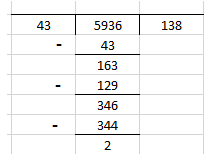Verification:

Dividend = divisor × quotient + remainder

5936 = 43 × 138 + 2

5936 = 43 × (100 + 38) + 2

= 4300 + 1634 + 2

= 5936

Therefore, verified.

#### Exercise 5(F) page no: 46

1. For each pattern, given below, write the next three steps:

(i) 1 × 9 + 1 = 10

12 × 9 + 2 = 110

123 × 9 + 3 = 1110

(ii) 9 × 9 + 7 = 88

98 ×9 + 6 = 888

987 ×9 + 5 = 8888

(iii) 1 × 8 + 1 = 9

12 × 8 + 2 = 98

123 × 8 + 3 = 987

(iv) 111 ÷ 3 = 37

222 ÷ 6 = 37

333 ÷ 9 = 37

Solution:

(i) 1 × 9 + 1 = 10

12 × 9 + 2 = 110

123 × 9 + 3 = 1110

1234 × 9 + 4 = 11110

12345 × 9 + 5 = 111110

123456 × 9 + 6 = 1111110

(ii) 9 × 9 + 7 = 88

98 × 9 + 6 = 888

987 × 9 + 5 = 8888

9876 × 9 + 4 = 88888

98765 × 9 + 3 = 888888

987654 × 9 + 2 = 8888888

(iii) 1 × 8 + 1 = 9

12 × 8 + 2 = 98

123 × 8 + 3 = 987

1234 × 8 + 4 = 9876

12345 × 8 + 5 = 98765

123456 × 8 + 6 = 987654

(iv) 111 ÷ 3 = 37

222 ÷ 6 = 37

333 ÷ 9 = 37

444 ÷ 12 = 37

555 ÷ 15 = 37

666 ÷ 18 = 37

2. Complete each of the following magic squares:

(i)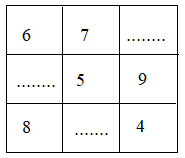(ii)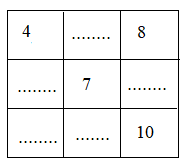(iii)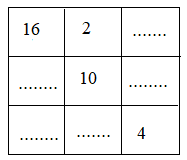Solution:

(i) Sum for row-wise is as follows

6 + 7 + 2 = 15

1 + 5 + 9 = 15

8 + 3 + 4 = 15

Sum for column wise is as follows

6 + 1 + 8 = 15

7 + 5 + 3 = 15

2 + 9 + 4 = 15

Sum for diagonal wise is as follows

6 + 5 + 4 = 15

2 + 5 + 8 = 15

Hence, the magic square is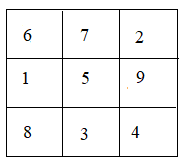(ii) Row wise sum is as follows:

4 + 9 + 8 = 21

11 + 7 + 3 = 21

6 + 5 + 10 = 21

Column wise sum is as follows

4 + 11 + 6 = 21

9 + 7 + 5 = 21

8 + 3 + 10 = 21

Diagonal wise sum is as follows

4 + 7 + 10 = 21

8 + 7 + 6 = 21

Hence, the magic square is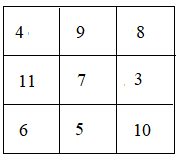(iii) Row wise sum is as follows

16 + 2 + 12 = 30

6 + 10 + 14 = 30

8 + 18 + 4 = 30

Column wise sum is as follows

16 + 6 + 8 = 30

2 + 10 + 18 = 30

12 + 14 + 4 = 30

Diagonal wise sum is as follows

16 + 10 + 4 = 30

12 + 10 + 8 = 30

Hence, the magic square is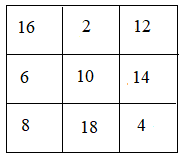3. See the following pattern carefully: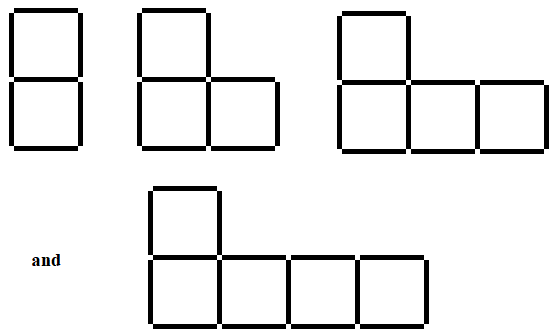(i) If n denotes the number of figures and S denotes the number of matchsticks; find S in terms of n.

(ii) Find how many matches are required to make the:

(1) 15th figure

(2) 40th figure

(iii) Write a description of the pattern in words,

Solution:

The table is

 n 1 2 3 4 S 7 10 13 16

S = 3n + 4

(ii) (1) 15th figure has = 3 × 15 + 4

= 49 matches

(2) 40th figure has = 3 × 40 + 4

= 124 matches

(iii) It is clear that each time the figure (n) is increased by 4, the number of matches (S) are increased by 3.

4. (i) In the following pattern, draw the next two figures.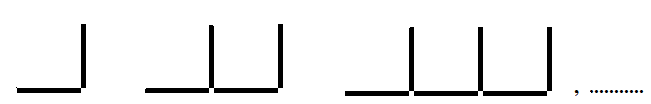(ii) Construct a table to describe the figures in the above pattern.

(iii) If n denotes the number of figures and L denotes the number of matchsticks, find L in terms of n.

(iv) Find how many matchsticks are required to make the:

(1) 12th figure

(2) 20th figure

Solution:

(i)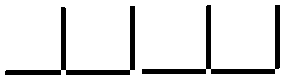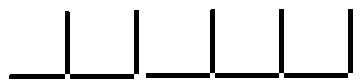(ii) The table is

 n 1 2 3 4 5 L 2 4 6 8 10

(iii) Hence, the value of L is

L = 2n

(iv) (1) Number of matchsticks in 12th figure = 2 × 12

= 24

(2) Number of matchsticks in 20th figure = 2 × 20

= 40

5. In each of the following patterns, construct next figure.

(i) In each case, if n denotes the number of figures and F denotes the number of matchsticks used, find F in terms of n.

(ii) Also find, in each case, how many matchsticks are required to make the:

16th figure and 30th figure.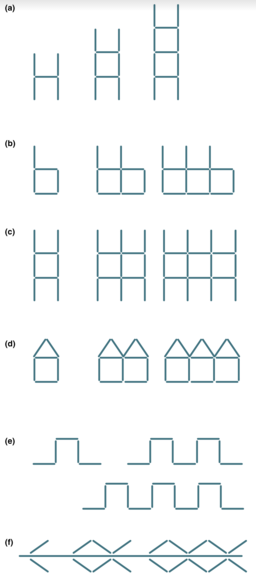Solution:

(a)(b)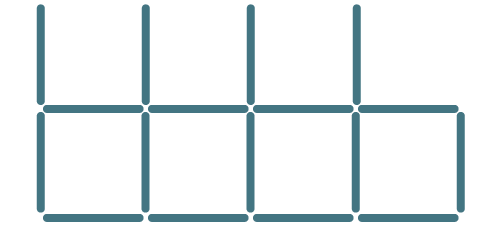(c)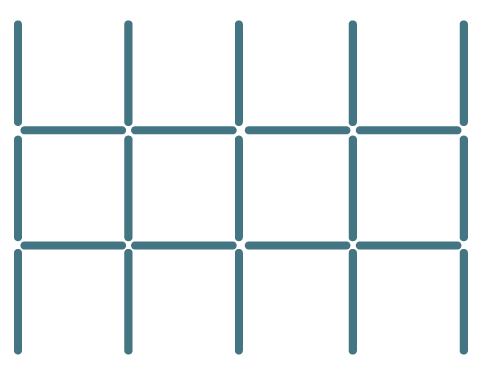(d)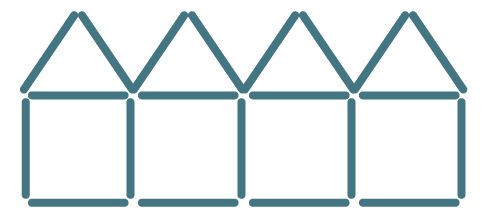(e)(f)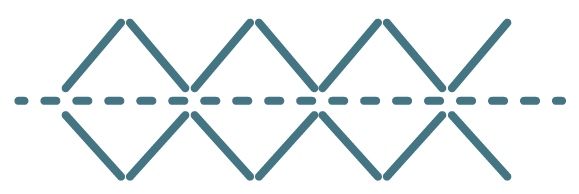(i) (a) F = 3n + 2

(b) F = 4n + 1

(c) F = 5n + 3

(d) F = 5n + 1

(e) F = 4n + 1

(f) F = 4n – 2

16th figure

(a) 3 × 16 + 2

= 48 + 2

= 50

(b) F = 4 × 16 + 1

= 64 + 1

= 65

(c) F = 5 × 16 + 3

= 80 + 3

= 83

(d) F = 5 × 16 + 1

= 80 + 1

= 81

(e) F = 4 × 16 + 1

= 64 + 1

= 65

(f) F = 4 × 16 – 2

= 64 – 2

= 62

30th figure

(a) F = 3 × 30 + 2

= 90 + 2

= 92

(b) F = 4 × 30 + 1

= 120 + 1

= 121

(c) F = 5 × 30 + 3

= 150 + 3

= 153

(d) F = 5 × 30 + 1

= 150 + 1

= 151

(e) F = 4 × 30 + 1

= 120 + 1

= 121

(f) F = 4 × 30 – 2

= 120 – 2

= 118